# NCERT Exemplar Solutions for Class 11 Maths Chapter 3 Trigonometric Functions

NCERT Exemplar Solutions Class 11 Maths Chapter 3 Trigonometric Functions are provided here. These NCERT Exemplar solutions are created by the BYJU’S expert faculty to help students in the preparation of their examinations. These expert faculty solve and provide the NCERT Exemplar Solution for Class 11, which would help students to solve the problems comfortably. They give a detailed and stepwise explanation of the problems given in the exercises in the NCERT Exemplar textbook for Class 11. In CBSE Class 11 Trigonometric Functions chapter, students are introduced to a lot of important topics, which will be helpful for those who wish to pursue Mathematics as a subject in further classes. These solutions help students prepare for their upcoming Board Exams by covering the whole syllabus, in accordance with the NCERT guidelines. The PDF for Chapter 3, exemplar problems and solutions can be downloaded and practised offline as well.

Chapter 3 of NCERT Exemplar Solutions for Class 11 Maths Trigonometric Functions explains domain and range of trigonometric functions. Trigonometric Functions can simply be defined as the functions of an angle. It is also called by many other names such as angle functions, circular functions, and goniometric functions. The major functions that are most commonly known to everybody are Sine, Cosine, and Tangent. Some functions such as infinite series and differential equations are used for whole numbers and complex numbers. In Chapter 3, students will learn and solve exemplar problems based on topics like;

• The relation between degree and radian
• Trigonometric functions
• Domain and range of trigonometric functions
• Sine, cosine, and tangent of some angles less than 90°
• Allied or related angles
• Functions of negative angles
• Some formulae regarding compound angles
• Trigonometric equations
• General Solution of Trigonometric Equations

## Download PDF Of NCERT Exemplar Class 10 Maths Solutions Chapter 3 – Trigonometric Functions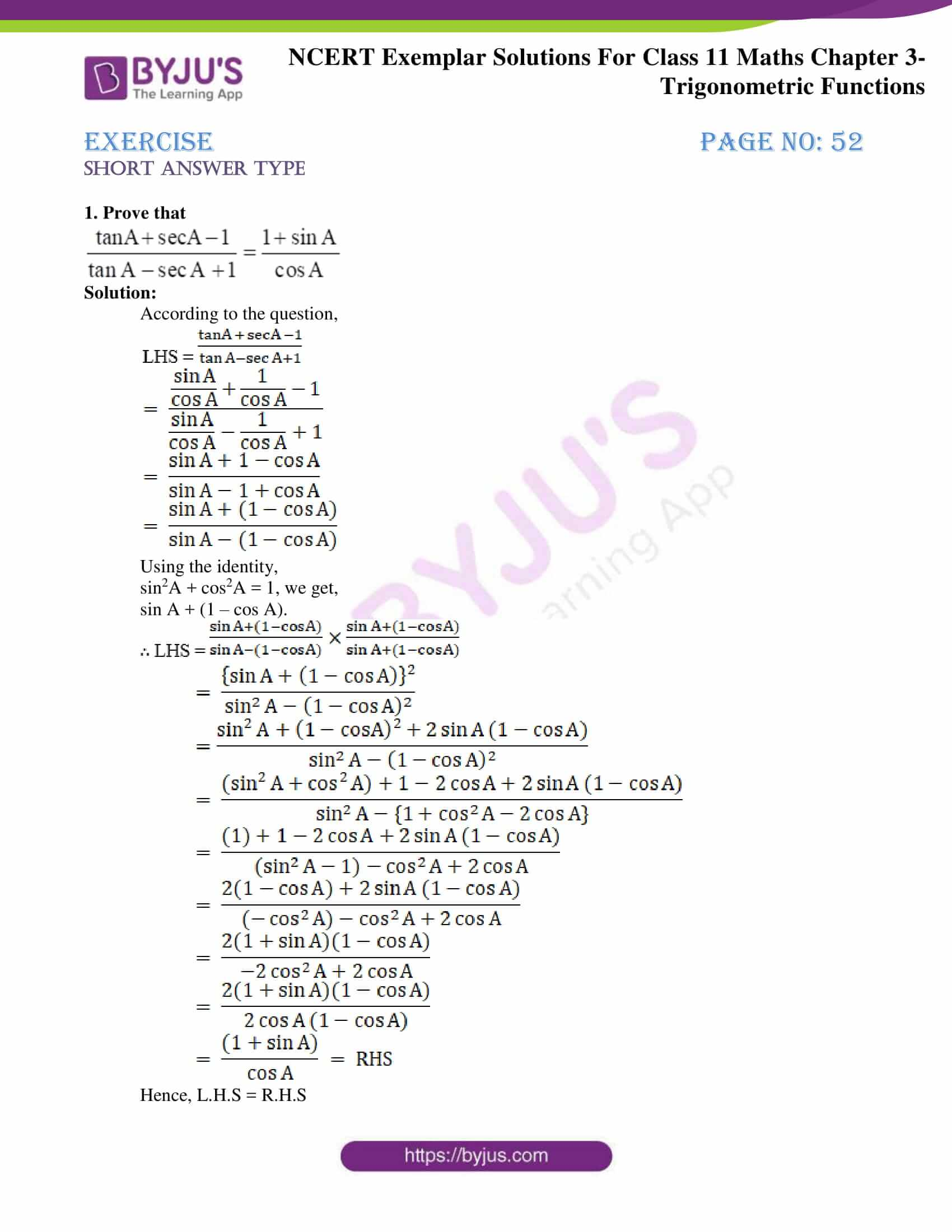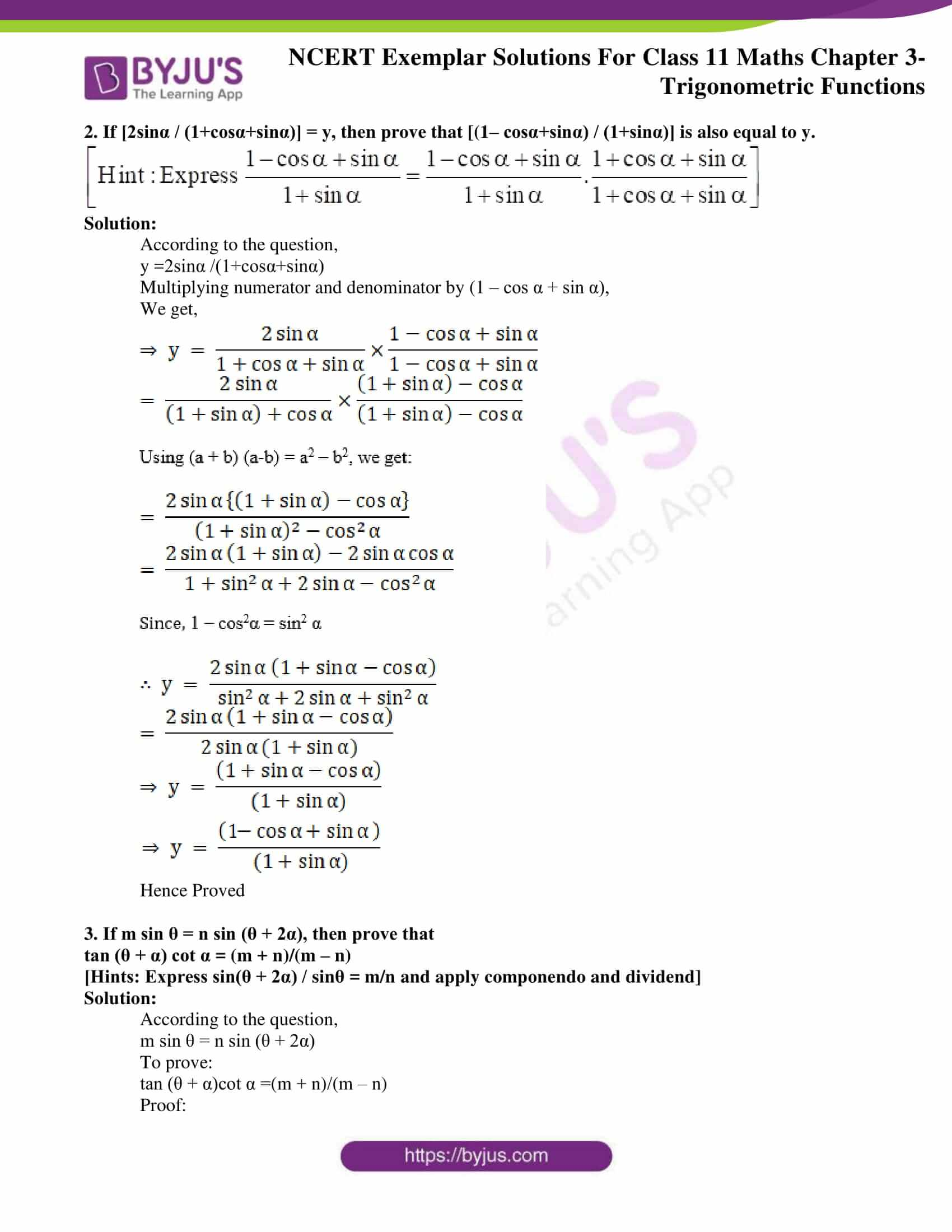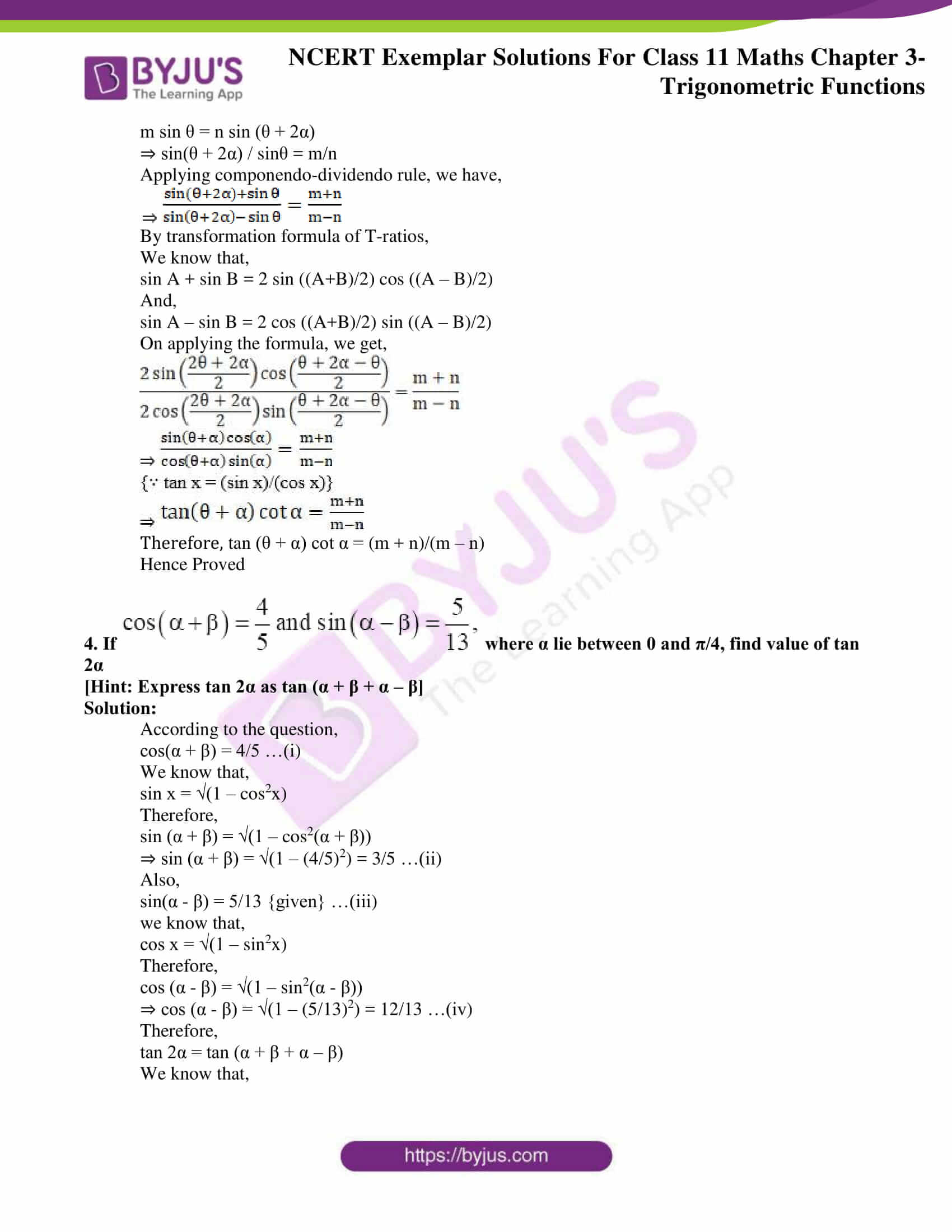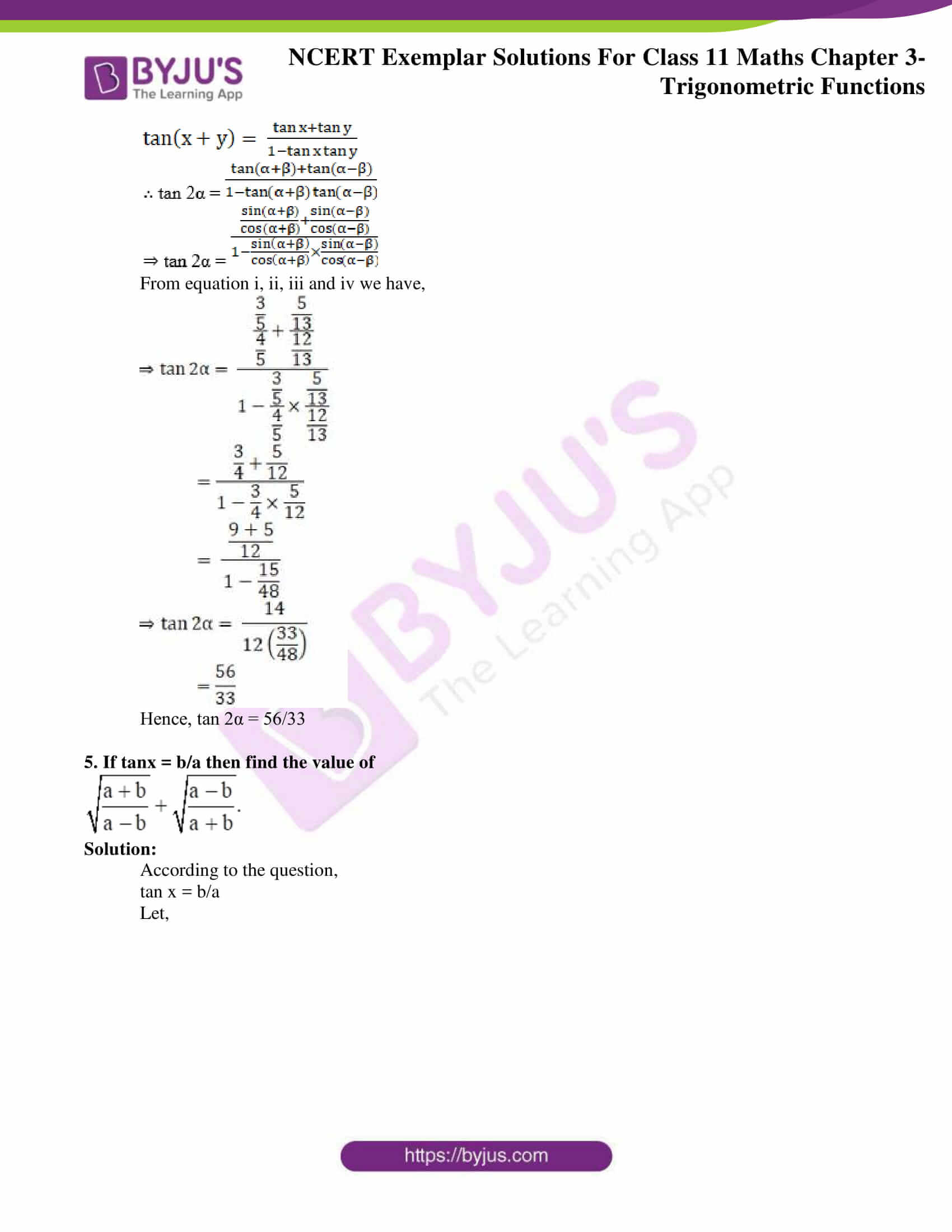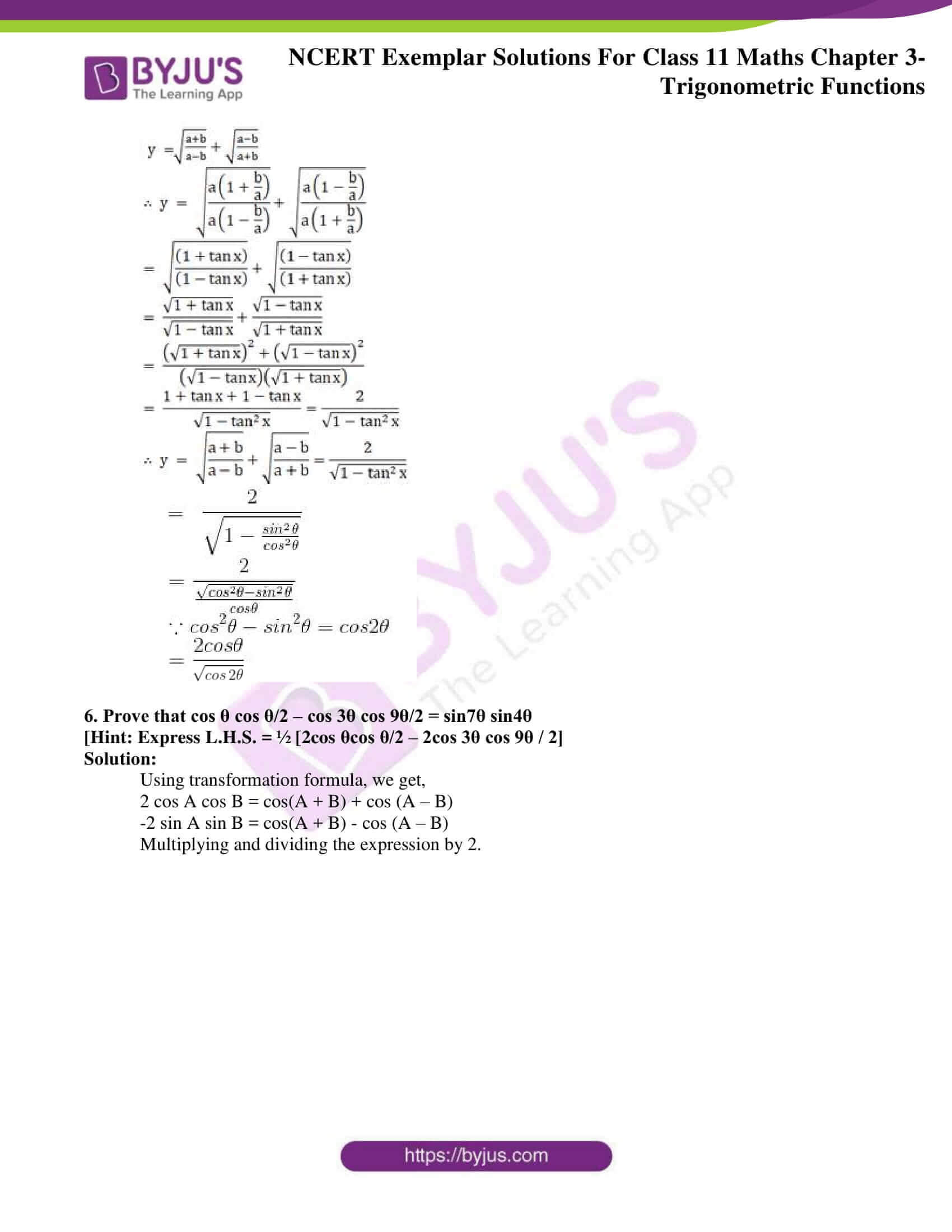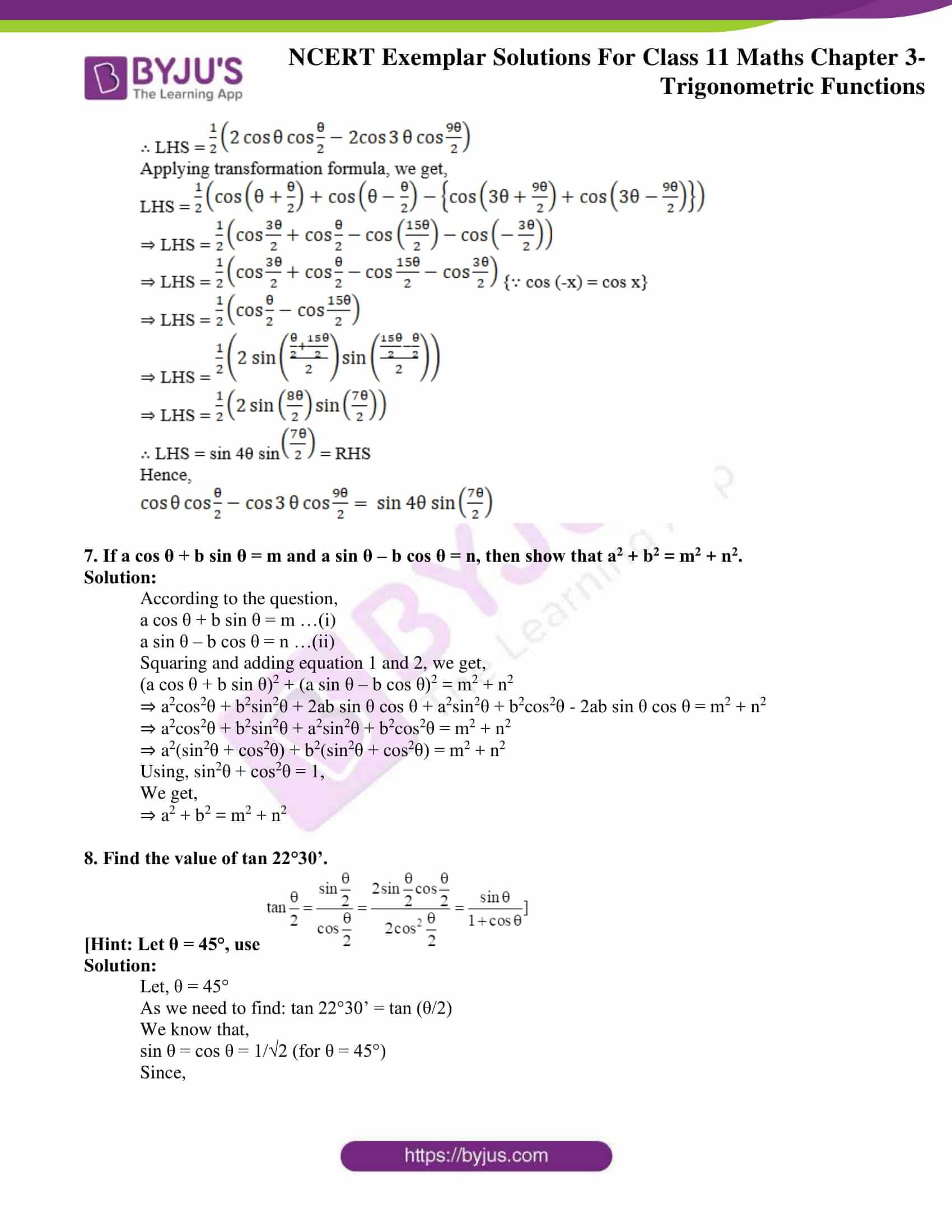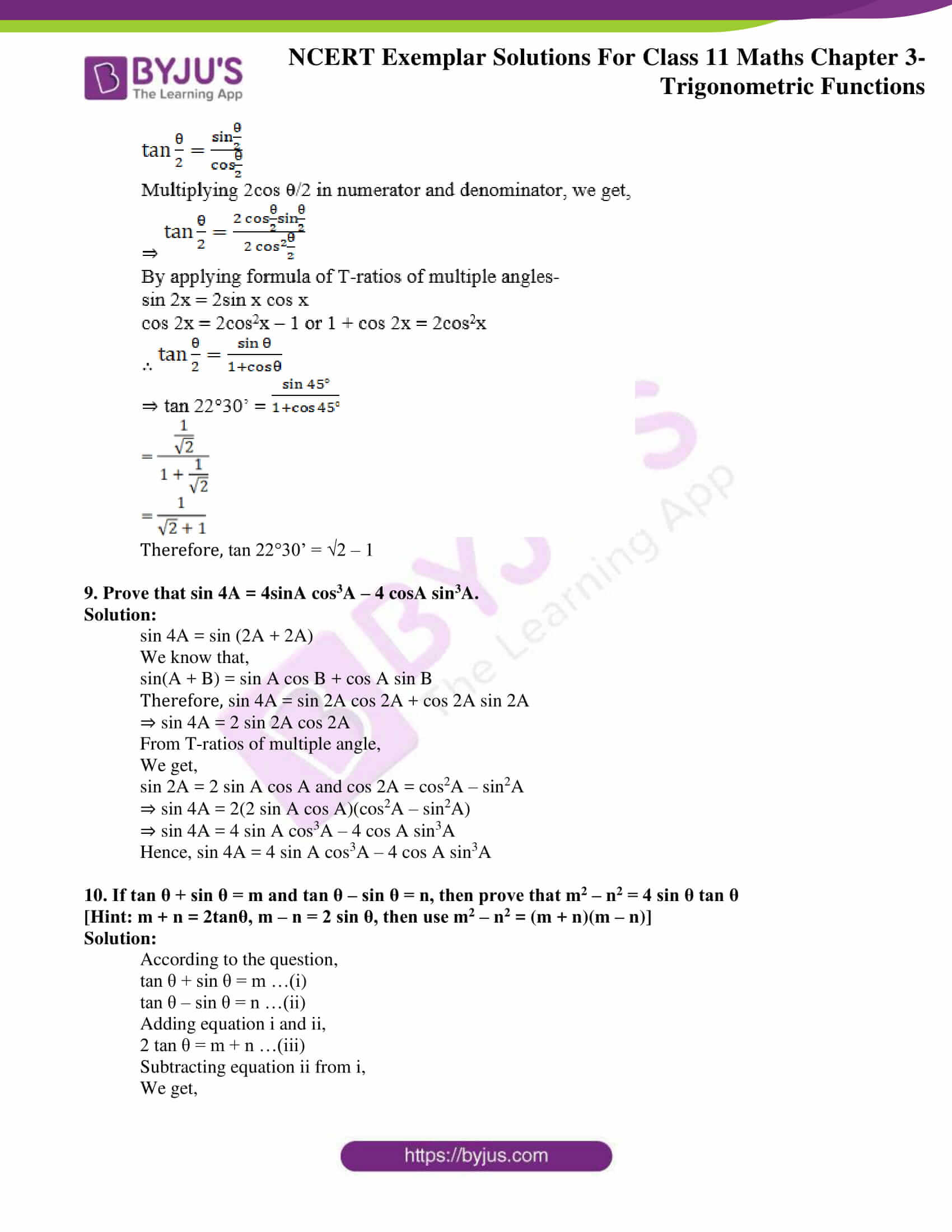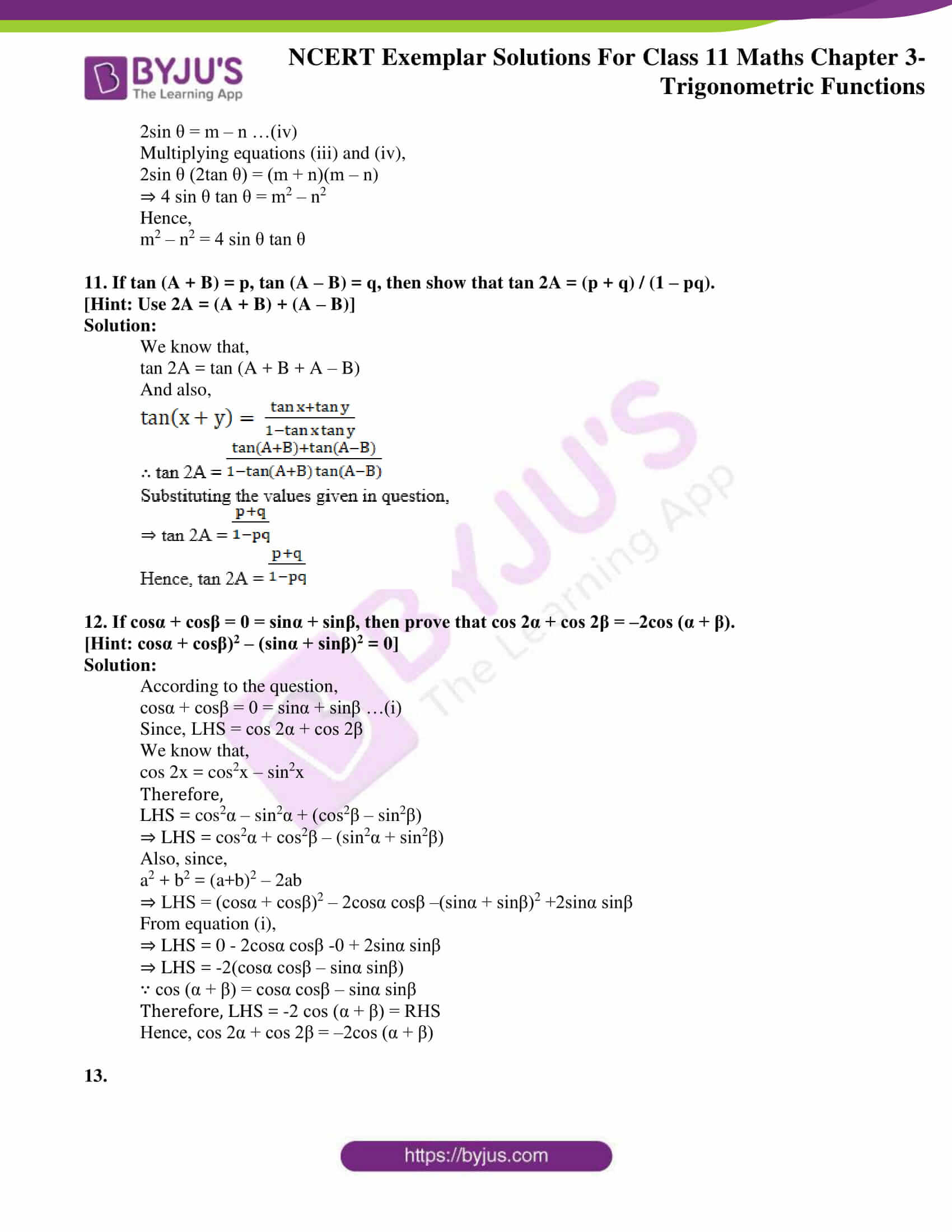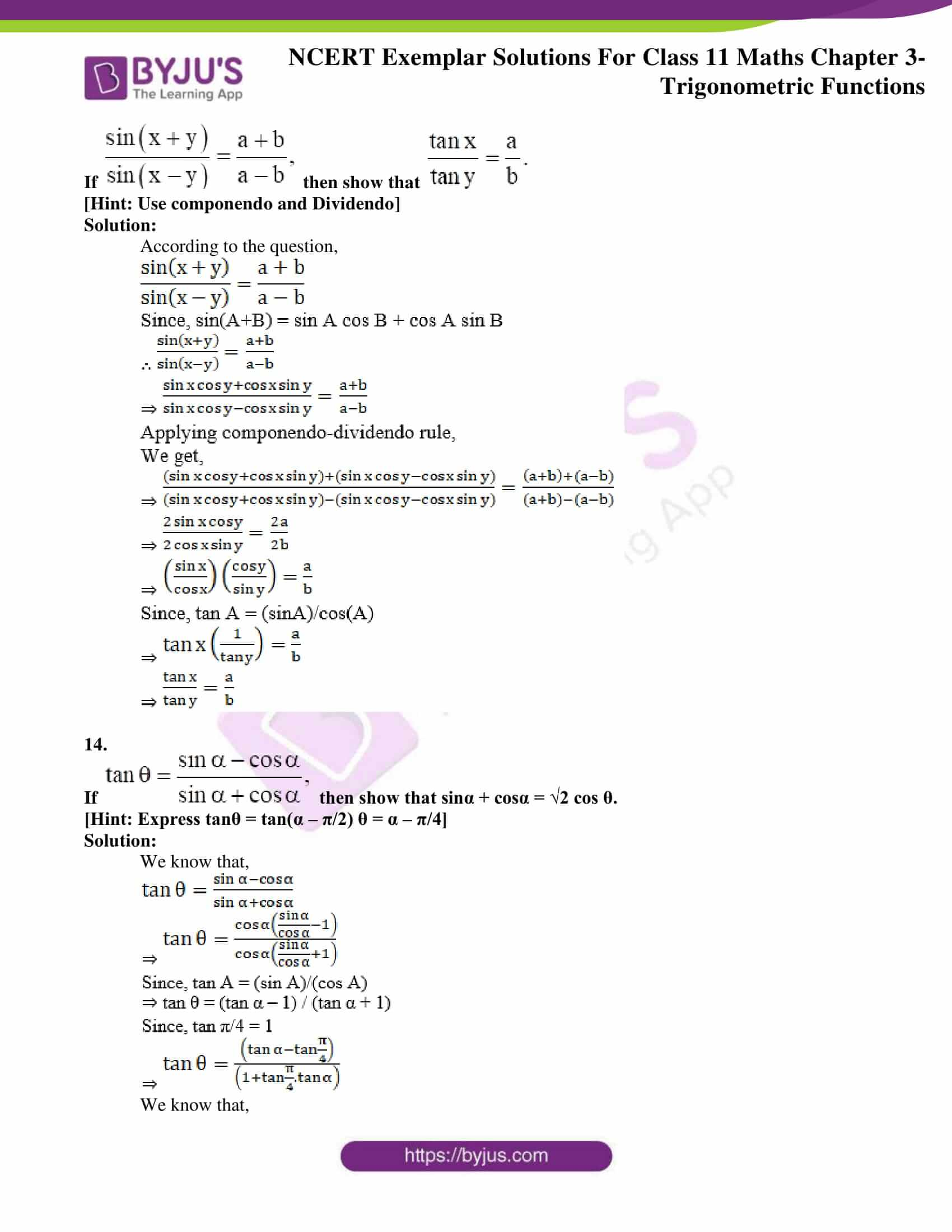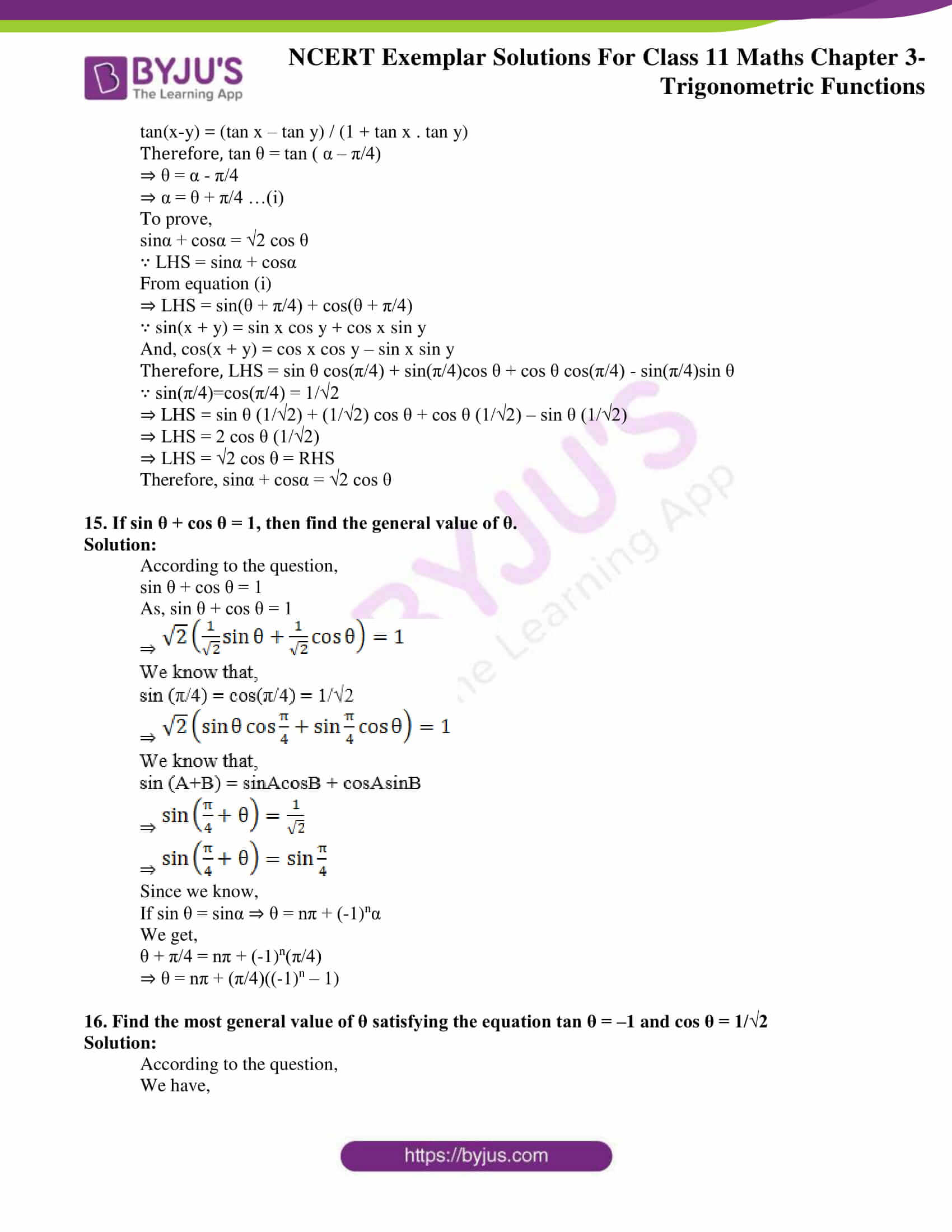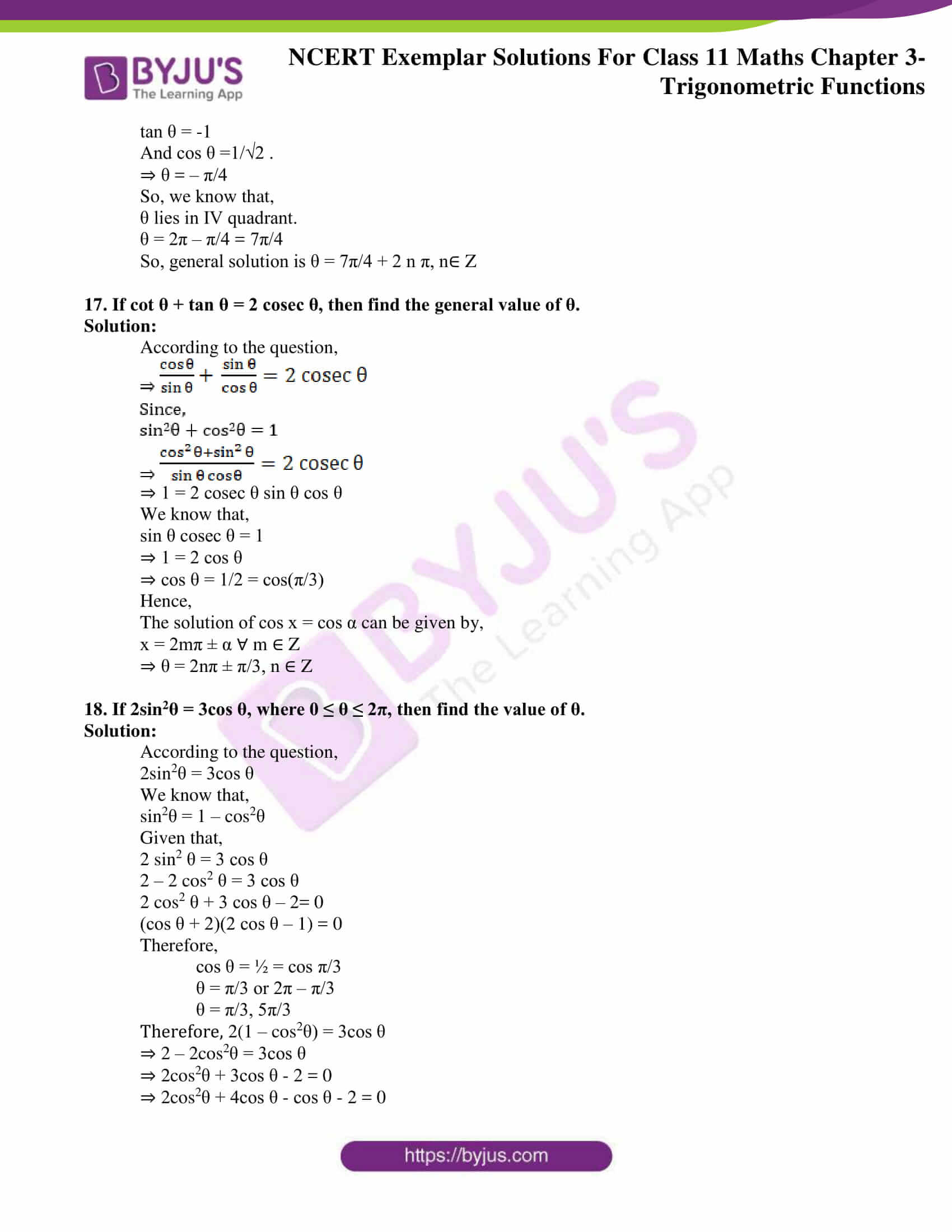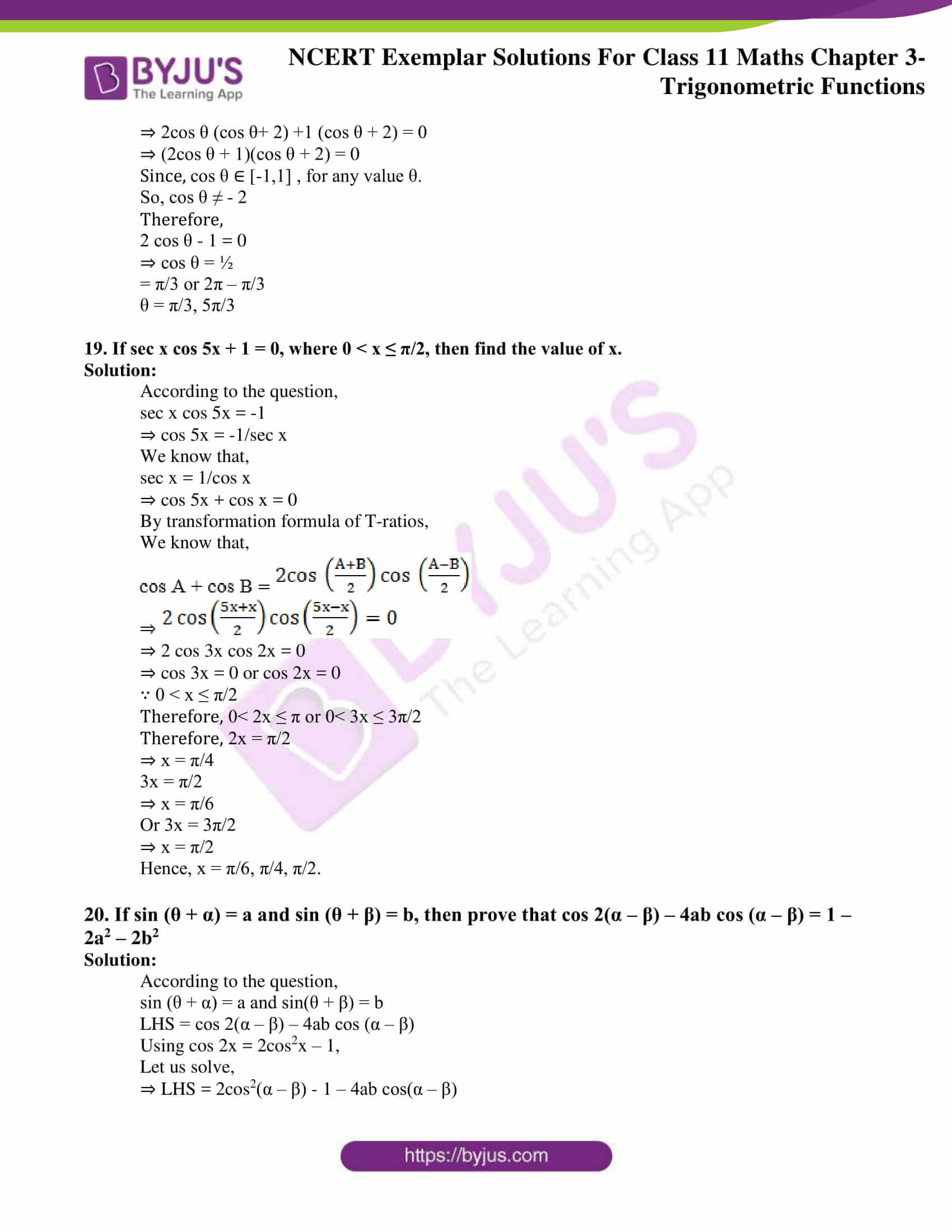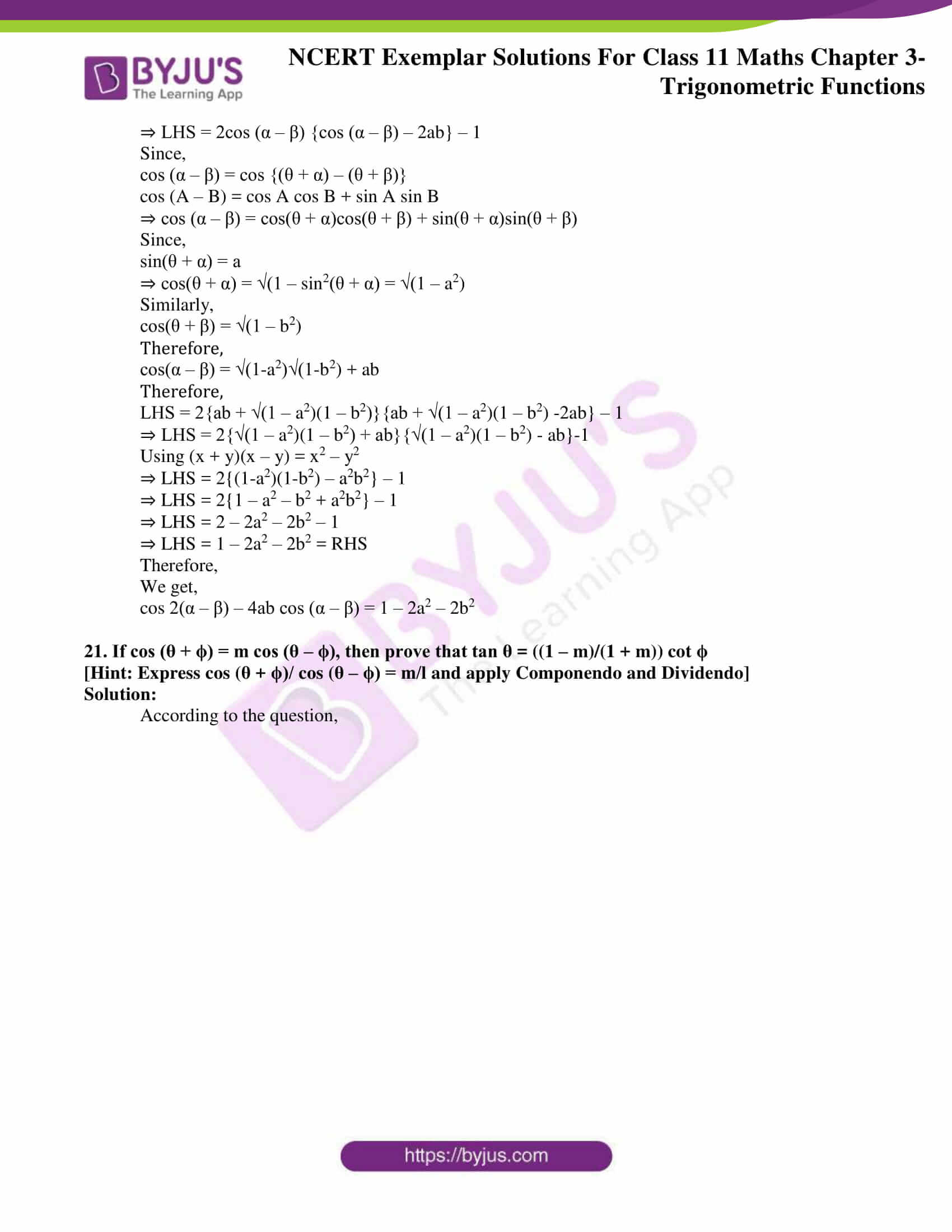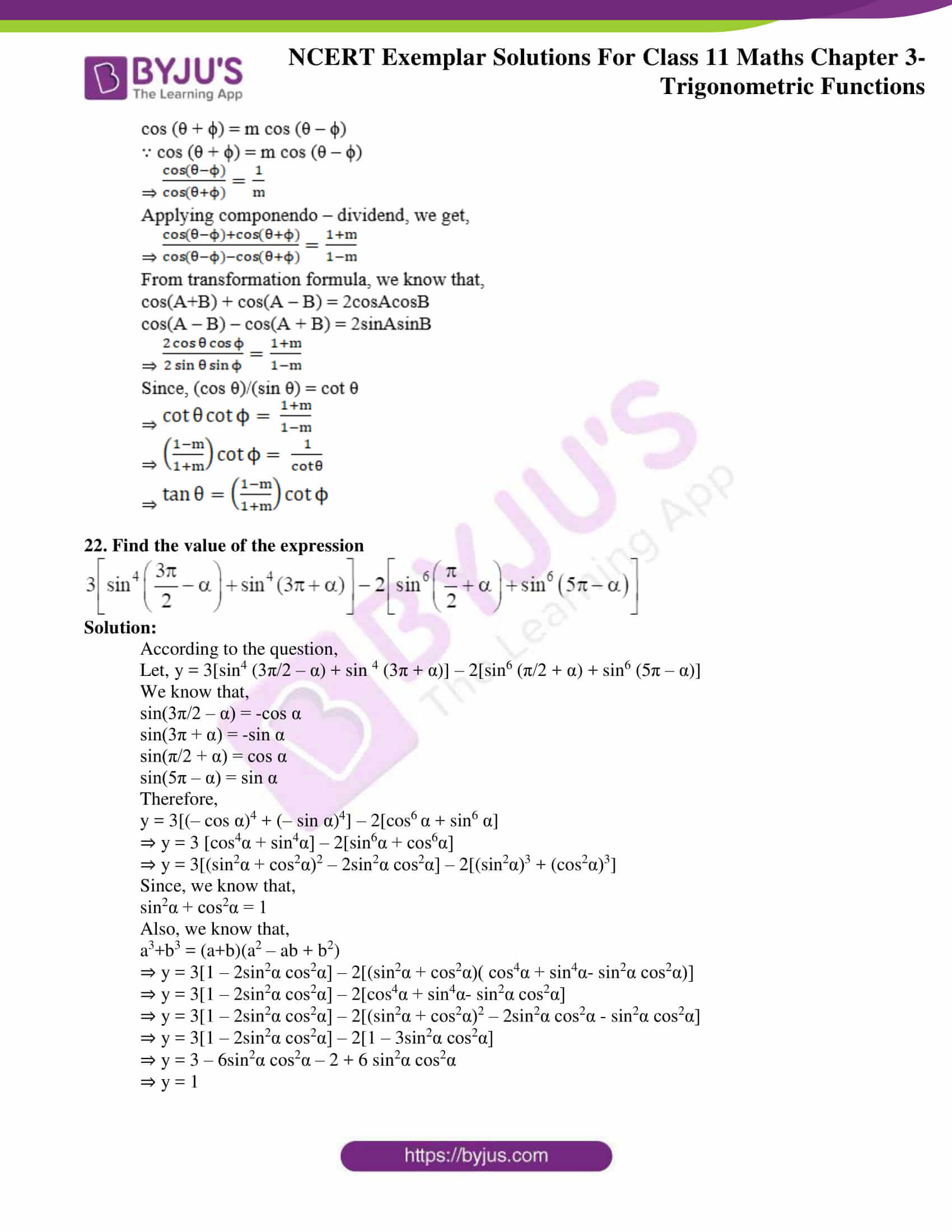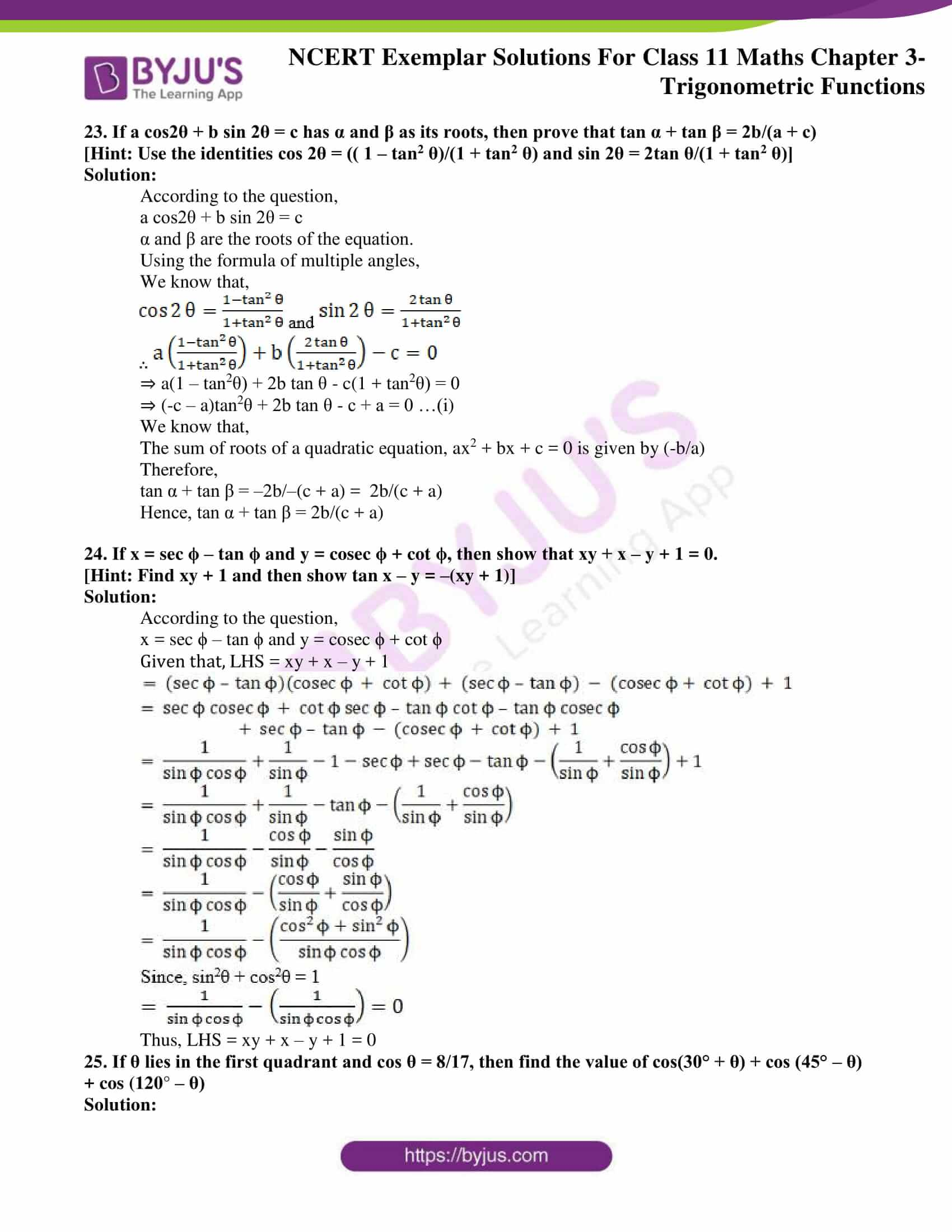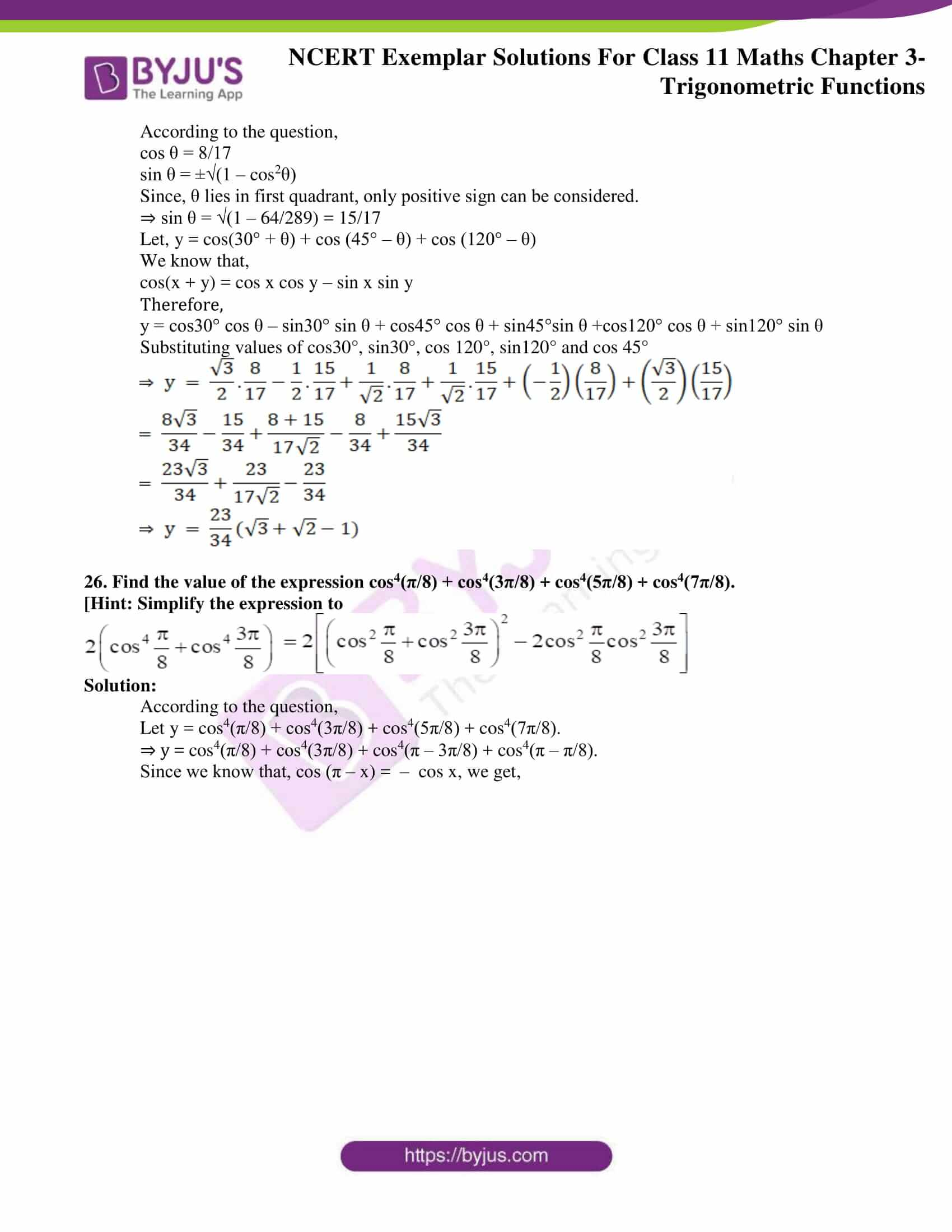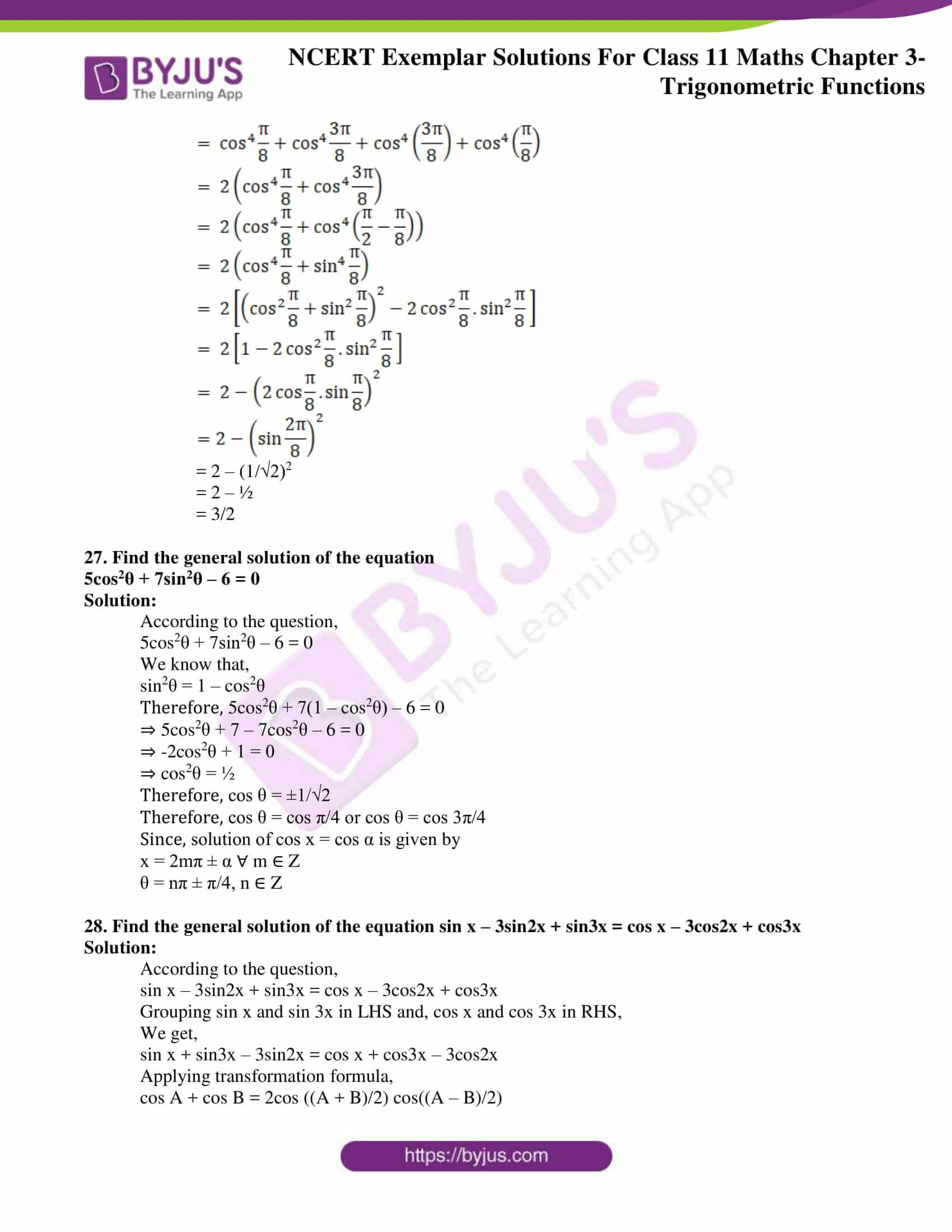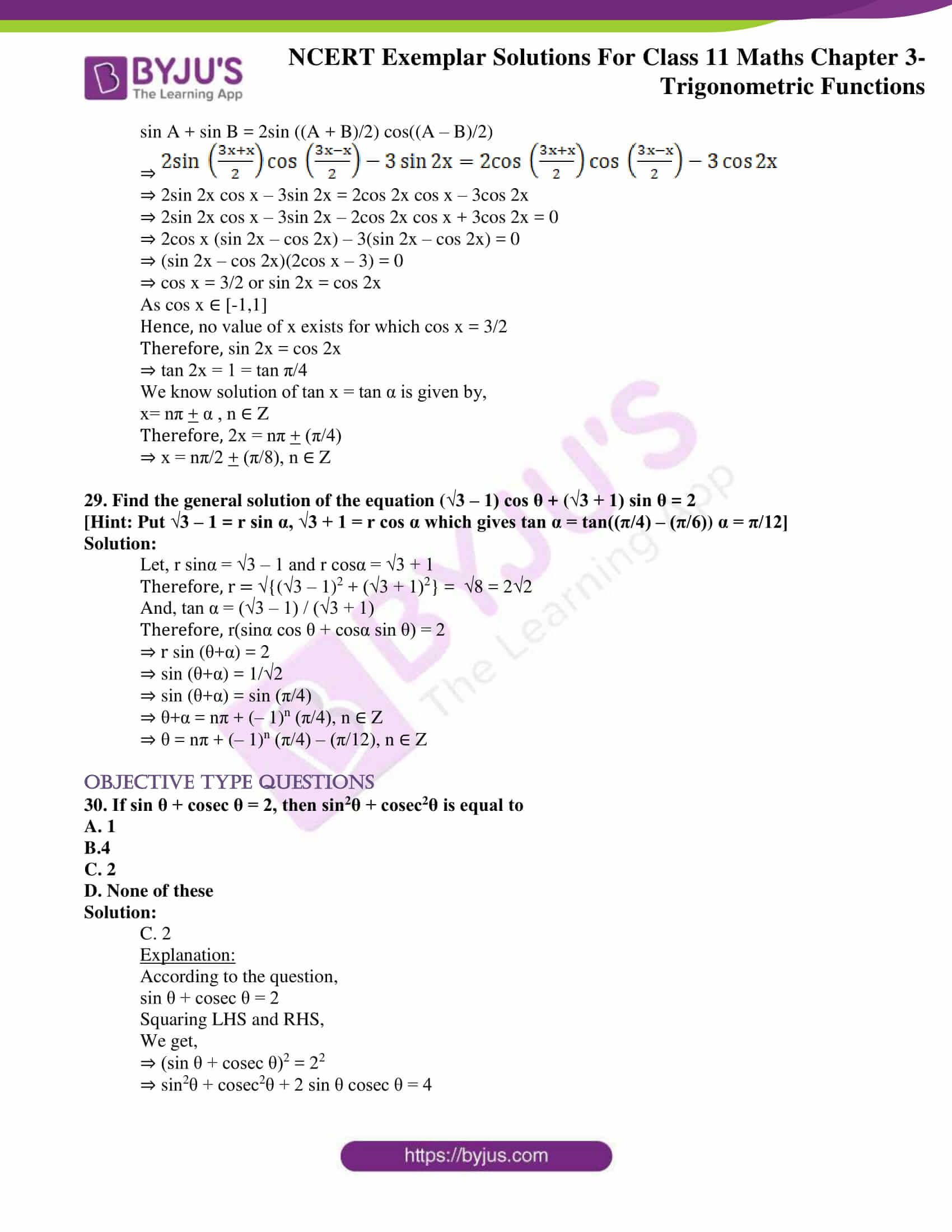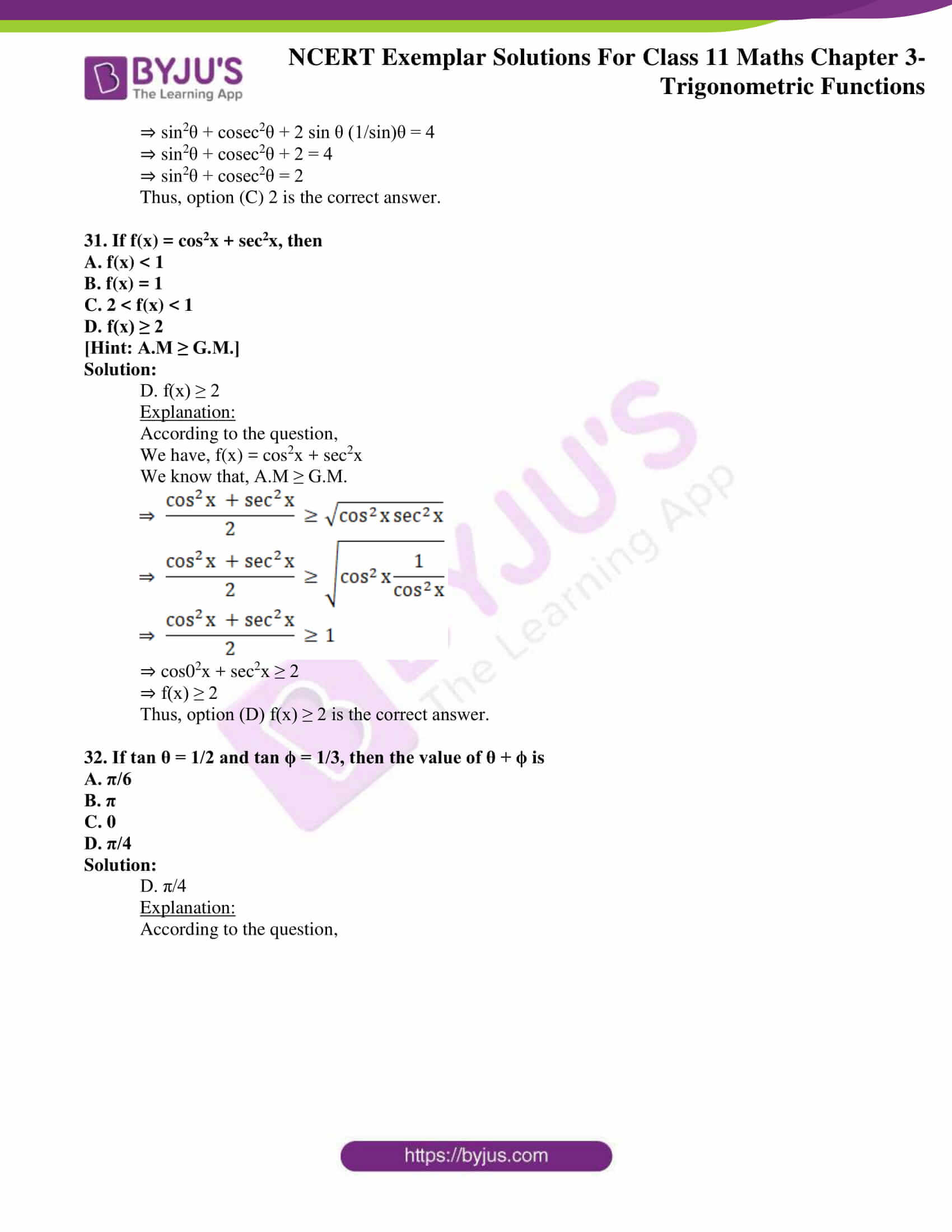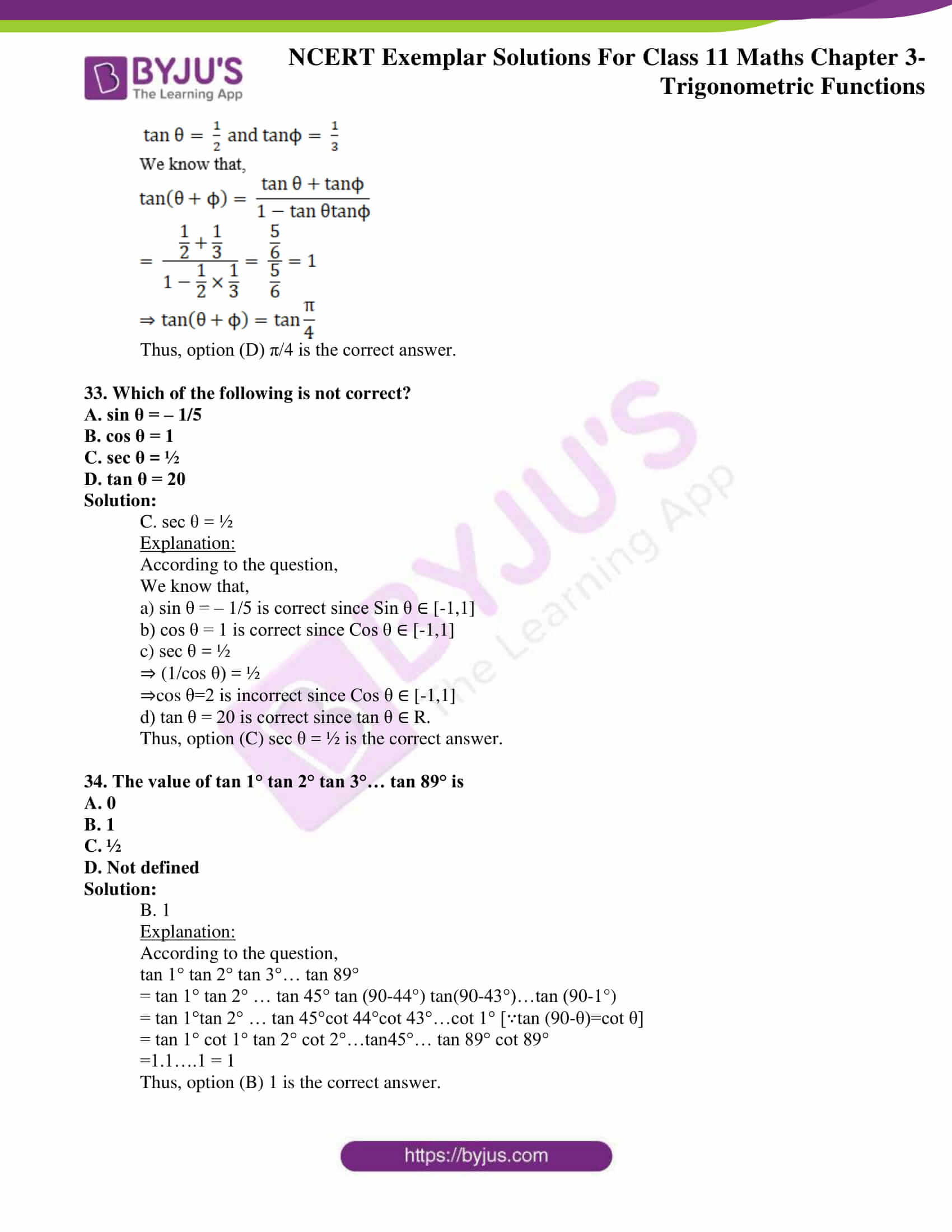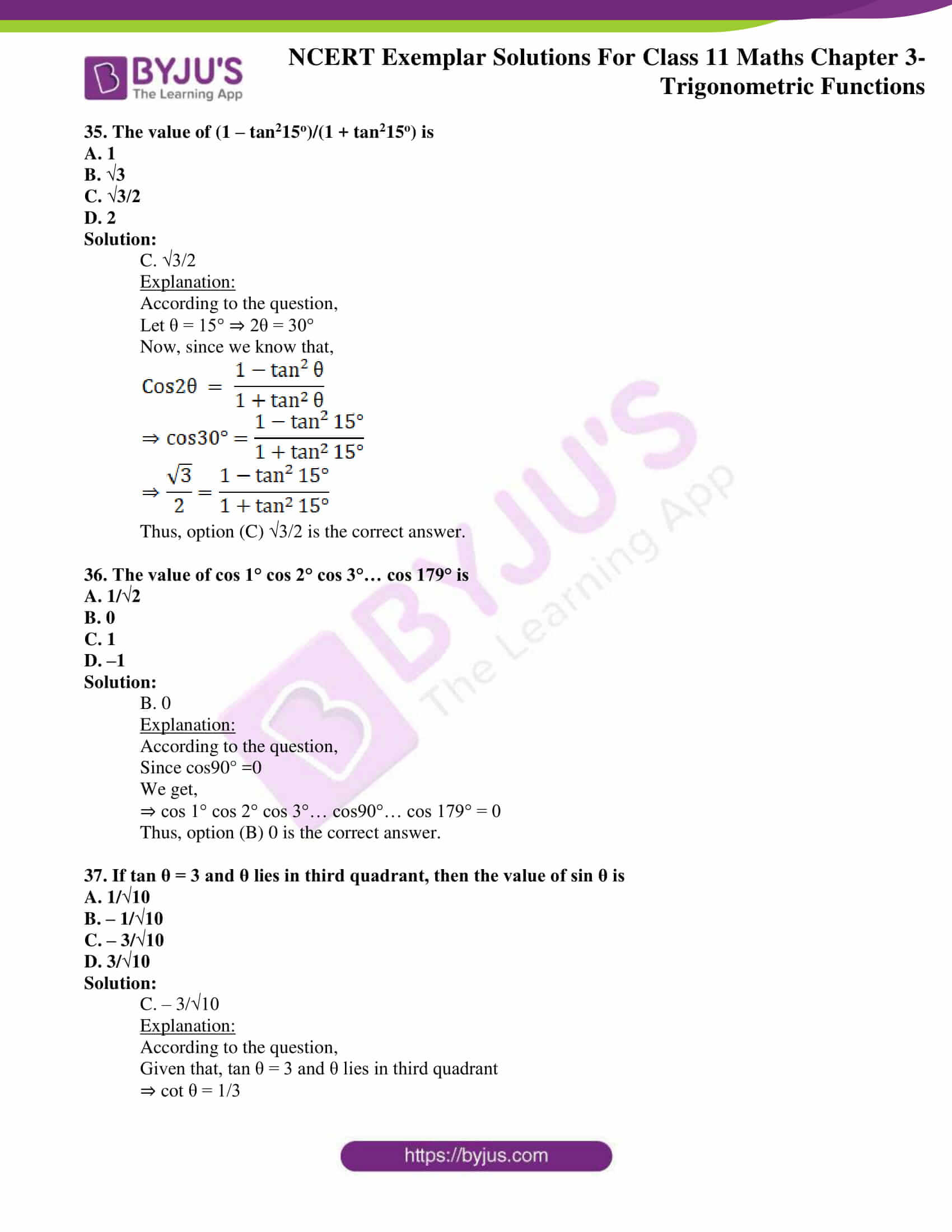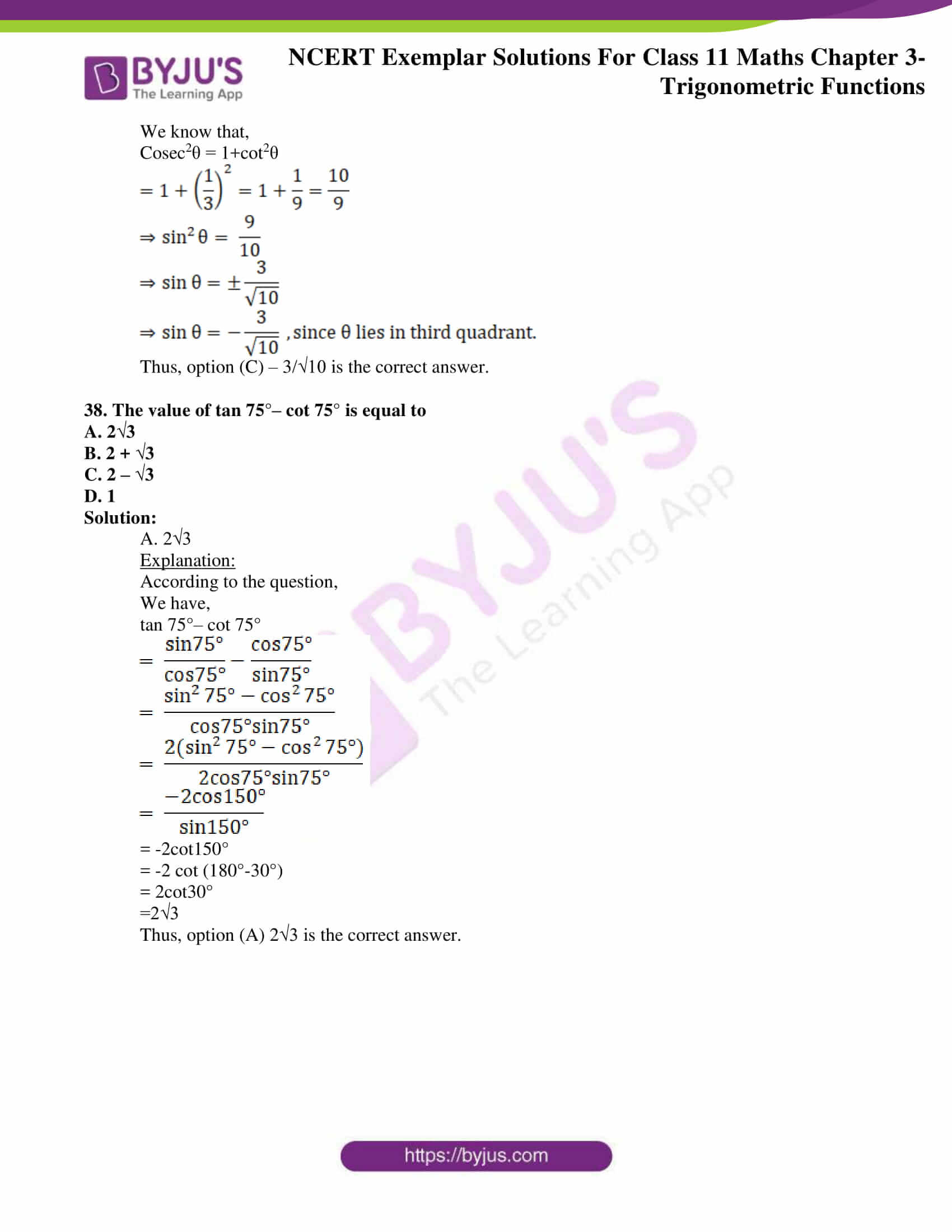### Access Answers to NCERT Exemplar Class 10 Maths Chapter 3 – Trigonometric Functions

Exercise Page No: 52

1. Prove that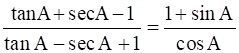Solution:

According to the question,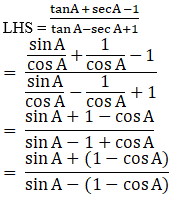Using the identity,

sin2A + cos2A = 1, we get,

sin A + (1 – cos A).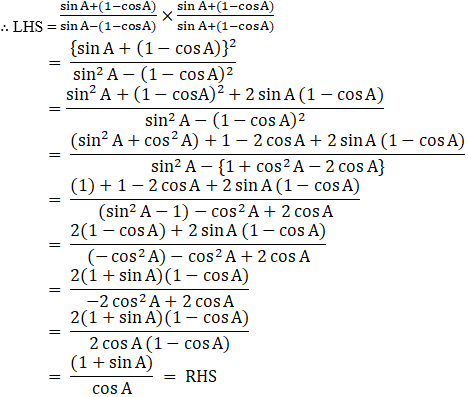Hence, L.H.S = R.H.S

2. If [2sinα / (1+cosα+sinα)] = y, then prove that [(1– cosα+sinα) / (1+sinα)] is also equal to y.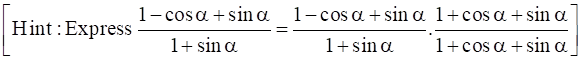Solution:

According to the question,

y =2sinα /(1+cosα+sinα)

Multiplying numerator and denominator by (1 – cos α + sin α),

We get,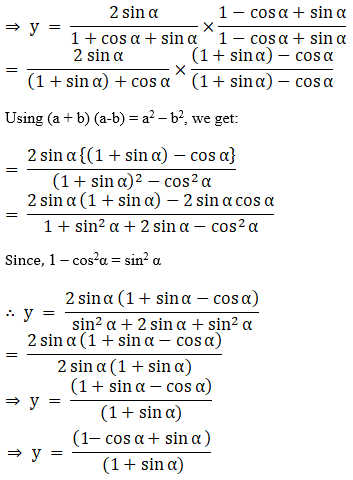Hence Proved

3. If m sin θ = n sin (θ + 2α), then prove that

tan (θ + α) cot α = (m + n)/(m – n)

[Hints: Express sin(θ + 2α) / sinθ = m/n and apply componendo and dividend]

Solution:

According to the question,

m sin θ = n sin (θ + 2α)

To prove:

tan (θ + α)cot α =(m + n)/(m – n)

Proof:

m sin θ = n sin (θ + 2α)

⇒ sin(θ + 2α) / sinθ = m/n

Applying componendo-dividendo rule, we have,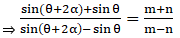By transformation formula of T-ratios,

We know that,

sin A + sin B = 2 sin ((A+B)/2) cos ((A – B)/2)

And,

sin A – sin B = 2 cos ((A+B)/2) sin ((A – B)/2)

On applying the formula, we get,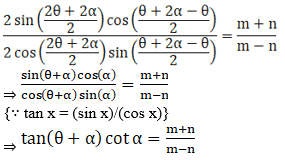Therefore, tan (θ + α) cot α = (m + n)/(m – n)

Hence Proved

4. If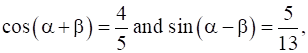where α lie between 0 and π/4, find value of tan 2α
[Hint: Express tan 2α as tan (α + β + α – β]

Solution:

According to the question,

cos(α + β) = 4/5 …(i)

We know that,

sin x = √(1 – cos2x)

Therefore,

sin (α + β) = √(1 – cos2(α + β))

⇒ sin (α + β) = √(1 – (4/5)2) = 3/5 …(ii)

Also,

sin(α – β) = 5/13 {given} …(iii)

we know that,

cos x = √(1 – sin2x)

Therefore,

cos (α – β) = √(1 – sin2(α – β))

⇒ cos (α – β) = √(1 – (5/13)2) = 12/13 …(iv)

Therefore,

tan 2α = tan (α + β + α – β)

We know that,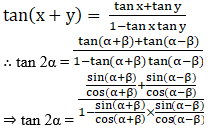From equation i, ii, iii and iv we have,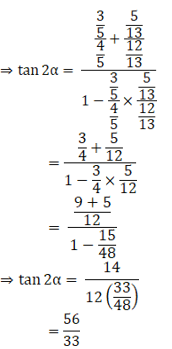Hence, tan 2α = 56/33

5. If tanx = b/a then find the value of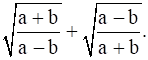Solution:

According to the question,

tan x = b/a

Let,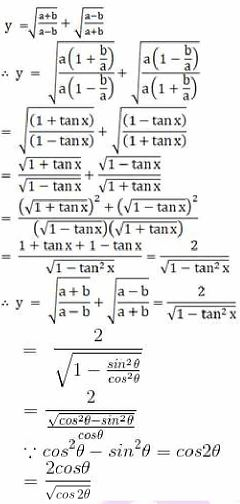6. Prove that cos θ cos θ/2 – cos 3θ cos 9θ/2 = sin7θ sin4θ
[Hint: Express L.H.S. = ½ [2cos θcos θ/2 – 2cos 3θ cos 9θ / 2]

Solution:

Using transformation formula, we get,

2 cos A cos B = cos(A + B) + cos (A – B)

-2 sin A sin B = cos(A + B) – cos (A – B)

Multiplying and dividing the expression by 2.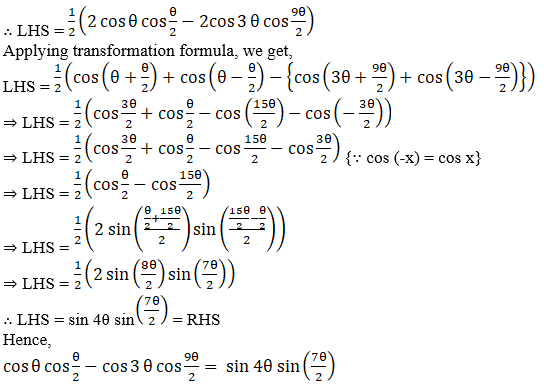7. If a cos θ + b sin θ = m and a sin θ – b cos θ = n, then show that a2 + b2 = m2 + n2.

Solution:

According to the question,

a cos θ + b sin θ = m …(i)

a sin θ – b cos θ = n …(ii)

Squaring and adding equation 1 and 2, we get,

(a cos θ + b sin θ)2 + (a sin θ – b cos θ)2 = m2 + n2

⇒ a2cos2θ + b2sin2θ + 2ab sin θ cos θ + a2sin2θ + b2cos2θ – 2ab sin θ cos θ = m2 + n2

⇒ a2cos2θ + b2sin2θ + a2sin2θ + b2cos2θ = m2 + n2

⇒ a2(sin2θ + cos2θ) + b2(sin2θ + cos2θ) = m2 + n2

Using, sin2θ + cos2θ = 1,

We get,

⇒ a2 + b2 = m2 + n2

8. Find the value of tan 22°30’.
[Hint: Let θ = 45°, use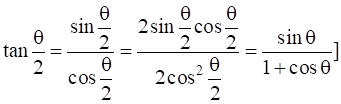Solution:

Let, θ = 45°

As we need to find: tan 22°30’ = tan (θ/2)

We know that,

sin θ = cos θ = 1/√2 (for θ = 45°)

Since,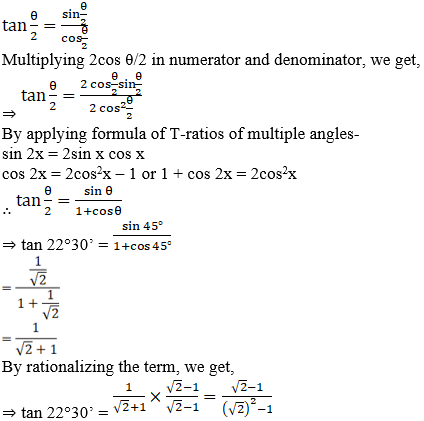Therefore, tan 22°30’ = √2 – 1

9. Prove that sin 4A = 4sinA cos3A – 4 cosA sin3A.

Solution:

sin 4A = sin (2A + 2A)

We know that,

sin(A + B) = sin A cos B + cos A sin B

Therefore, sin 4A = sin 2A cos 2A + cos 2A sin 2A

⇒ sin 4A = 2 sin 2A cos 2A

From T-ratios of multiple angle,

We get,

sin 2A = 2 sin A cos A and cos 2A = cos2A – sin2A

⇒ sin 4A = 2(2 sin A cos A)(cos2A – sin2A)

⇒ sin 4A = 4 sin A cos3A – 4 cos A sin3A

Hence, sin 4A = 4 sin A cos3A – 4 cos A sin3A

10. If tan θ + sin θ = m and tan θ – sin θ = n, then prove that m2 – n2 = 4 sin θ tan θ

[Hint: m + n = 2tanθ, m – n = 2 sin θ, then use m2 – n2 = (m + n)(m – n)]

Solution:

According to the question,

tan θ + sin θ = m …(i)

tan θ – sin θ = n …(ii)

2 tan θ = m + n …(iii)

Subtracting equation ii from i,

We get,

2sin θ = m – n …(iv)

Multiplying equations (iii) and (iv),

2sin θ (2tan θ) = (m + n)(m – n)

⇒ 4 sin θ tan θ = m2 – n2

Hence,

m2 – n2 = 4 sin θ tan θ

11. If tan (A + B) = p, tan (A – B) = q, then show that tan 2A = (p + q) / (1 – pq).
[Hint: Use 2A = (A + B) + (A – B)]

Solution:

We know that,

tan 2A = tan (A + B + A – B)

And also,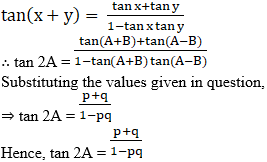12. If cosα + cosβ = 0 = sinα + sinβ, then prove that cos 2α + cos 2β = –2cos (α + β).
[Hint: cosα + cosβ)2 – (sinα + sinβ)2 = 0]

Solution:

According to the question,

cosα + cosβ = 0 = sinα + sinβ …(i)

Since, LHS = cos 2α + cos 2β

We know that,

cos 2x = cos2x – sin2x

Therefore,

LHS = cos2α – sin2α + (cos2β – sin2β)

⇒ LHS = cos2α + cos2β – (sin2α + sin2β)

Also, since,

a2 + b2 = (a+b)2 – 2ab

⇒ LHS = (cosα + cosβ)2 – 2cosα cosβ –(sinα + sinβ)2 +2sinα sinβ

From equation (i),

⇒ LHS = 0 – 2cosα cosβ -0 + 2sinα sinβ

⇒ LHS = -2(cosα cosβ – sinα sinβ)

∵ cos (α + β) = cosα cosβ – sinα sinβ

Therefore, LHS = -2 cos (α + β) = RHS

Hence, cos 2α + cos 2β = –2cos (α + β)

13.

If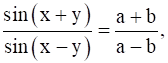then show that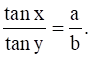[Hint: Use componendo and Dividendo]

Solution:

According to the question,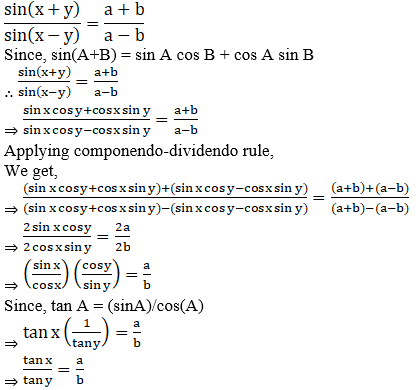14.

If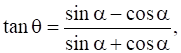then show that sinα + cosα = √2 cos θ.
[Hint: Express tanθ = tan(α – π/2) θ = α – π/4]

Solution:

We know that,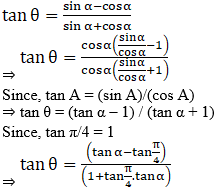We know that,

tan(x-y) = (tan x – tan y) / (1 + tan x . tan y)

Therefore, tan θ = tan ( α – π/4)

⇒ θ = α – π/4

⇒ α = θ + π/4 …(i)

To prove,

sinα + cosα = √2 cos θ

∵ LHS = sinα + cosα

From equation (i)

⇒ LHS = sin(θ + π/4) + cos(θ + π/4)

∵ sin(x + y) = sin x cos y + cos x sin y

And, cos(x + y) = cos x cos y – sin x sin y

Therefore, LHS = sin θ cos(π/4) + sin(π/4)cos θ + cos θ cos(π/4) – sin(π/4)sin θ

∵ sin(π/4)=cos(π/4) = 1/√2

⇒ LHS = sin θ (1/√2) + (1/√2) cos θ + cos θ (1/√2) – sin θ (1/√2)

⇒ LHS = 2 cos θ (1/√2)

⇒ LHS = √2 cos θ = RHS

Therefore, sinα + cosα = √2 cos θ

15. If sin θ + cos θ = 1, then find the general value of θ.

Solution:

According to the question,

sin θ + cos θ = 1

As, sin θ + cos θ = 1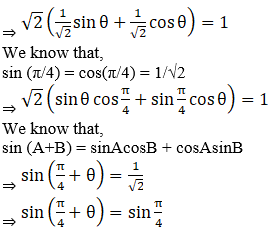Since we know,

If sin θ = sinα ⇒ θ = nπ + (-1)nα

We get,

θ + π/4 = nπ + (-1)n(π/4)

⇒ θ = nπ + (π/4)((-1)n – 1)

16. Find the most general value of θ satisfying the equation tan θ = –1 and cos θ = 1/√2

Solution:

According to the question,

We have,

tan θ = -1

And cos θ =1/√2 .

⇒ θ = – π/4

So, we know that,

θ = 2π – π/4 = 7π/4

So, general solution is θ = 7π/4 + 2 n π, n∈ Z

17. If cot θ + tan θ = 2 cosec θ, then find the general value of θ.

Solution:

According to the question,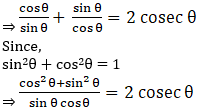⇒ 1 = 2 cosec θ sin θ cos θ

We know that,

sin θ cosec θ = 1

⇒ 1 = 2 cos θ

⇒ cos θ = 1/2 = cos(π/3)

Hence,

The solution of cos x = cos α can be given by,

x = 2mπ ± α ∀ m ∈ Z

⇒ θ = 2nπ ± π/3, n ∈ Z

18. If 2sin2θ = 3cos θ, where 0 ≤ θ ≤ 2π, then find the value of θ.

Solution:

According to the question,

2sin2θ = 3cos θ

We know that,

sin2θ = 1 – cos2θ

Given that,

2 sin2 θ = 3 cos θ

2 – 2 cos2 θ = 3 cos θ

2 cos2 θ + 3 cos θ – 2= 0

(cos θ + 2)(2 cos θ – 1) = 0

Therefore,

cos θ = ½ = cos π/3

θ = π/3 or 2π – π/3

θ = π/3, 5π/3

Therefore, 2(1 – cos2θ) = 3cos θ

⇒ 2 – 2cos2θ = 3cos θ

⇒ 2cos2θ + 3cos θ – 2 = 0

⇒ 2cos2θ + 4cos θ – cos θ – 2 = 0

⇒ 2cos θ (cos θ+ 2) +1 (cos θ + 2) = 0

⇒ (2cos θ + 1)(cos θ + 2) = 0

Since, cos θ ∈ [-1,1] , for any value θ.

So, cos θ ≠ – 2

Therefore,

2 cos θ – 1 = 0

⇒ cos θ = ½

= π/3 or 2π – π/3

θ = π/3, 5π/3

19. If sec x cos 5x + 1 = 0, where 0 < x ≤ π/2, then find the value of x.

Solution:

According to the question,

sec x cos 5x = -1

⇒ cos 5x = -1/sec x

We know that,

sec x = 1/cos x

⇒ cos 5x + cos x = 0

By transformation formula of T-ratios,

We know that,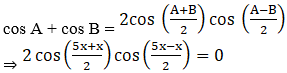⇒ 2 cos 3x cos 2x = 0

⇒ cos 3x = 0 or cos 2x = 0

∵ 0 < x ≤ π/2

Therefore, 0< 2x ≤ π or 0< 3x ≤ 3π/2

Therefore, 2x = π/2

⇒ x = π/4

3x = π/2

⇒ x = π/6

Or 3x = 3π/2

⇒ x = π/2

Hence, x = π/6, π/4, π/2.

20. If sin (θ + α) = a and sin (θ + β) = b, then prove that cos 2(α – β) – 4ab cos (α – β) = 1 – 2a2 – 2b2

Solution:

According to the question,

sin (θ + α) = a and sin(θ + β) = b

LHS = cos 2(α – β) – 4ab cos (α – β)

Using cos 2x = 2cos2x – 1,

Let us solve,

⇒ LHS = 2cos2(α – β) – 1 – 4ab cos(α – β)

⇒ LHS = 2cos (α – β) {cos (α – β) – 2ab} – 1

Since,

cos (α – β) = cos {(θ + α) – (θ + β)}

cos (A – B) = cos A cos B + sin A sin B

⇒ cos (α – β) = cos(θ + α)cos(θ + β) + sin(θ + α)sin(θ + β)

Since,

sin(θ + α) = a

⇒ cos(θ + α) = √(1 – sin2(θ + α) = √(1 – a2)

Similarly,

cos(θ + β) = √(1 – b2)

Therefore,

cos(α – β) = √(1-a2)√(1-b2) + ab

Therefore,

LHS = 2{ab + √(1 – a2)(1 – b2)}{ab + √(1 – a2)(1 – b2) -2ab} – 1

⇒ LHS = 2{√(1 – a2)(1 – b2) + ab}{√(1 – a2)(1 – b2) – ab}-1

Using (x + y)(x – y) = x2 – y2

⇒ LHS = 2{(1-a2)(1-b2) – a2b2} – 1

⇒ LHS = 2{1 – a2 – b2 + a2b2} – 1

⇒ LHS = 2 – 2a2 – 2b2 – 1

⇒ LHS = 1 – 2a2 – 2b2 = RHS

Therefore,

We get,

cos 2(α – β) – 4ab cos (α – β) = 1 – 2a2 – 2b2

21. If cos (θ + ϕ) = m cos (θ – ϕ), then prove that tan θ = ((1 – m)/(1 + m)) cot ϕ
[Hint: Express cos (θ + ϕ)/ cos (θ – ϕ) = m/l and apply Componendo and Dividendo] Solution:

According to the question,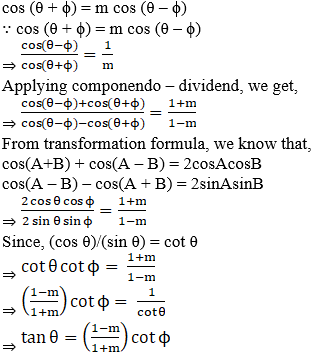22. Find the value of the expression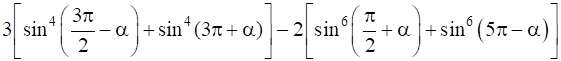Solution:

According to the question,

Let, y = 3[sin4 (3π/2 – α) + sin 4 (3π + α)] – 2[sin6 (π/2 + α) + sin6 (5π – α)]

We know that,

sin(3π/2 – α) = -cos α

sin(3π + α) = -sin α

sin(π/2 + α) = cos α

sin(5π – α) = sin α

Therefore,

y = 3[(– cos α)4 + (– sin α)4] – 2[cos6 α + sin6 α]

⇒ y = 3 [cos4α + sin4α] – 2[sin6α + cos6α]

⇒ y = 3[(sin2α + cos2α)2 – 2sin2α cos2α] – 2[(sin2α)3 + (cos2α)3]

Since, we know that,

sin2α + cos2α = 1

Also, we know that,

a3+b3 = (a+b)(a2 – ab + b2)

⇒ y = 3[1 – 2sin2α cos2α] – 2[(sin2α + cos2α)( cos4α + sin4α- sin2α cos2α)]

⇒ y = 3[1 – 2sin2α cos2α] – 2[cos4α + sin4α- sin2α cos2α]

⇒ y = 3[1 – 2sin2α cos2α] – 2[(sin2α + cos2α)2 – 2sin2α cos2α – sin2α cos2α]

⇒ y = 3[1 – 2sin2α cos2α] – 2[1 – 3sin2α cos2α]

⇒ y = 3 – 6sin2α cos2α – 2 + 6 sin2α cos2α

⇒ y = 1

23. If a cos2θ + b sin 2θ = c has α and β as its roots, then prove that tan α + tan β = 2b/(a + c)

[Hint: Use the identities cos 2θ = (( 1 – tan2 θ)/(1 + tan2 θ) and sin 2θ = 2tan θ/(1 + tan2 θ)]

Solution:

According to the question,

a cos2θ + b sin 2θ = c

α and β are the roots of the equation.

Using the formula of multiple angles,

We know that,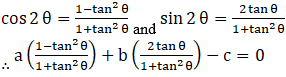⇒ a(1 – tan2θ) + 2b tan θ – c(1 + tan2θ) = 0

⇒ (-c – a)tan2θ + 2b tan θ – c + a = 0 …(i)

We know that,

The sum of roots of a quadratic equation, ax2 + bx + c = 0 is given by (-b/a)

Therefore,

tan α + tan β = –2b/–(c + a) = 2b/(c + a)

Hence, tan α + tan β = 2b/(c + a)

24. If x = sec ϕ – tan ϕ and y = cosec ϕ + cot ϕ, then show that xy + x – y + 1 = 0.

[Hint: Find xy + 1 and then show tan x – y = –(xy + 1)]

Solution:

According to the question,

x = sec ϕ – tan ϕ and y = cosec ϕ + cot ϕ

Given that, LHS = xy + x – y + 1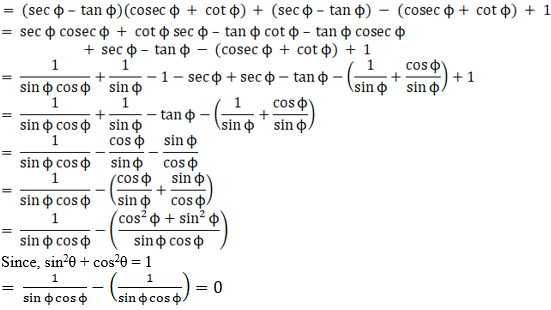Thus, LHS = xy + x – y + 1 = 0

25. If θ lies in the first quadrant and cos θ = 8/17, then find the value of cos(30° + θ) + cos (45° – θ) + cos (120° – θ)

Solution:

According to the question,

cos θ = 8/17

sin θ = ±√(1 – cos2θ)

Since, θ lies in first quadrant, only positive sign can be considered.

⇒ sin θ = √(1 – 64/289) = 15/17

Let, y = cos(30° + θ) + cos (45° – θ) + cos (120° – θ)

We know that,

cos(x + y) = cos x cos y – sin x sin y

Therefore,

y = cos30° cos θ – sin30° sin θ + cos45° cos θ + sin45°sin θ +cos120° cos θ + sin120° sin θ

Substituting values of cos30°, sin30°, cos 120°, sin120° and cos 45°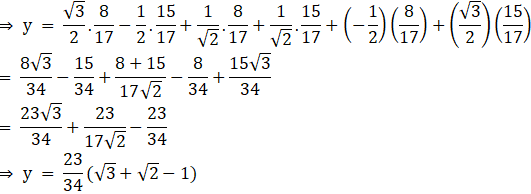26. Find the value of the expression cos4(π/8) + cos4(3π/8) + cos4(5π/8) + cos4(7π/8).
[Hint: Simplify the expression to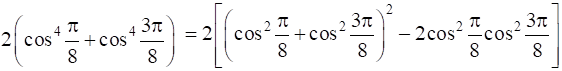Solution:

According to the question,

Let y = cos4(π/8) + cos4(3π/8) + cos4(5π/8) + cos4(7π/8).

⇒ y = cos4(π/8) + cos4(3π/8) + cos4(π – 3π/8) + cos4(π – π/8).

Since we know that, cos (π – x) = – cos x, we get,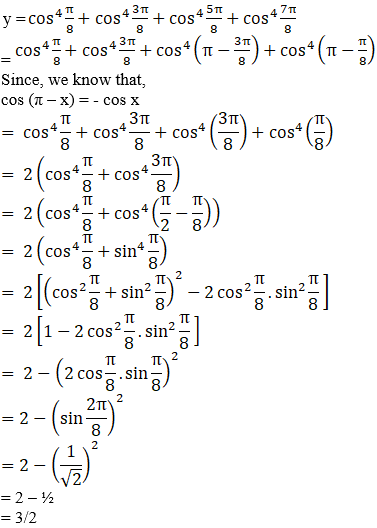= 2 – (1/√2)2

= 2 – ½

= 3/2

27. Find the general solution of the equation

5cos2θ + 7sin2θ – 6 = 0

Solution:

According to the question,

5cos2θ + 7sin2θ – 6 = 0

We know that,

sin2θ = 1 – cos2θ

Therefore, 5cos2θ + 7(1 – cos2θ) – 6 = 0

⇒ 5cos2θ + 7 – 7cos2θ – 6 = 0

⇒ -2cos2θ + 1 = 0

⇒ cos2θ = ½

Therefore, cos θ = ±1/√2

Therefore, cos θ = cos π/4 or cos θ = cos 3π/4

Since, solution of cos x = cos α is given by

x = 2mπ ± α ∀ m ∈ Z

θ = nπ ± π/4, n ∈ Z

28. Find the general solution of the equation sin x – 3sin2x + sin3x = cos x – 3cos2x + cos3x

Solution:

According to the question,

sin x – 3sin2x + sin3x = cos x – 3cos2x + cos3x

Grouping sin x and sin 3x in LHS and, cos x and cos 3x in RHS,

We get,

sin x + sin3x – 3sin2x = cos x + cos3x – 3cos2x

Applying transformation formula,

cos A + cos B = 2cos ((A + B)/2) cos((A – B)/2)

sin A + sin B = 2sin ((A + B)/2) cos((A – B)/2)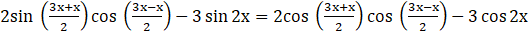⇒ 2sin 2x cos x – 3sin 2x = 2cos 2x cos x – 3cos 2x

⇒ 2sin 2x cos x – 3sin 2x – 2cos 2x cos x + 3cos 2x = 0

⇒ 2cos x (sin 2x – cos 2x) – 3(sin 2x – cos 2x) = 0

⇒ (sin 2x – cos 2x)(2cos x – 3) = 0

⇒ cos x = 3/2 or sin 2x = cos 2x

As cos x ∈ [-1,1]

Hence, no value of x exists for which cos x = 3/2

Therefore, sin 2x = cos 2x

⇒ tan 2x = 1 = tan π/4

We know solution of tan x = tan α is given by,

x= nπ + α , n ∈ Z

Therefore, 2x = nπ + (π/4)

⇒ x = nπ/2 + (π/8), n ∈ Z

29. Find the general solution of the equation (√3 – 1) cos θ + (√3 + 1) sin θ = 2

[Hint: Put √3 – 1 = r sin α, √3 + 1 = r cos α which gives tan α = tan((π/4) – (π/6)) α = π/12]

Solution:

Let, r sinα = √3 – 1 and r cosα = √3 + 1

Therefore, r = √{(√3 – 1)2 + (√3 + 1)2} = √8 = 2√2

And, tan α = (√3 – 1) / (√3 + 1)

Therefore, r(sinα cos θ + cosα sin θ) = 2

⇒ r sin (θ+α) = 2

⇒ sin (θ+α) = 1/√2

⇒ sin (θ+α) = sin (π/4)

⇒ θ+α = nπ + (– 1)n (π/4), n ∈ Z

⇒ θ = nπ + (– 1)n (π/4) – (π/12), n ∈ Z

Objective Type Questions

30. If sin θ + cosec θ = 2, then sin2θ + cosec2θ is equal to
A. 1

B.4
C. 2
D. None of these

Solution:

C. 2

Explanation:

According to the question,

sin θ + cosec θ = 2

Squaring LHS and RHS,

We get,

⇒ (sin θ + cosec θ)2 = 22

⇒ sin2θ + cosec2θ + 2 sin θ cosec θ = 4

⇒ sin2θ + cosec2θ + 2 sin θ (1/sin)θ = 4

⇒ sin2θ + cosec2θ + 2 = 4

⇒ sin2θ + cosec2θ = 2

Thus, option (C) 2 is the correct answer.

31. If f(x) = cos2x + sec2x, then
A. f(x) < 1
B. f(x) = 1
C. 2 < f(x) < 1
D. f(x) ≥ 2
[Hint: A.M ≥ G.M.]

Solution:

D. f(x) ≥ 2

Explanation:

According to the question,

We have, f(x) = cos2x + sec2x

We know that, A.M ≥ G.M.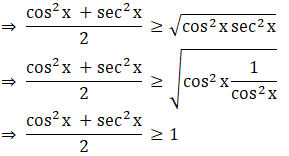⇒ cos02x + sec2x ≥ 2

⇒ f(x) ≥ 2

Thus, option (D) f(x) ≥ 2 is the correct answer.

32. If tan θ = 1/2 and tan ϕ = 1/3, then the value of θ + ϕ is
A. π/6
B. π
C. 0
D. π/4

Solution:

D. π/4

Explanation:

According to the question,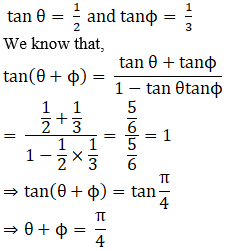Thus, option (D) π/4 is the correct answer.

33. Which of the following is not correct?
A. sin θ = – 1/5
B. cos θ = 1
C. sec θ = ½
D. tan θ = 20

Solution:

C. sec θ = ½

Explanation:

According to the question,

We know that,

a) sin θ = – 1/5 is correct since Sin θ ∈ [-1,1]

b) cos θ = 1 is correct since Cos θ ∈ [-1,1]

c) sec θ = ½

⇒ (1/cos θ) = ½

⇒cos θ=2 is incorrect since Cos θ ∈ [-1,1]

d) tan θ = 20 is correct since tan θ ∈ R.

Thus, option (C) sec θ = ½ is the correct answer.

34. The value of tan 1° tan 2° tan 3°… tan 89° is
A. 0
B. 1
C. ½
D. Not defined

Solution:

B. 1

Explanation:

According to the question,

tan 1° tan 2° tan 3°… tan 89°

= tan 1° tan 2° … tan 45° tan (90-44°) tan(90-43°)…tan (90-1°)

= tan 1°tan 2° … tan 45°cot 44°cot 43°…cot 1° [∵tan (90-θ)=cot θ]

= tan 1° cot 1° tan 2° cot 2°…tan45°… tan 89° cot 89°

=1.1….1 = 1

Thus, option (B) 1 is the correct answer.

35. The value of (1 – tan215o)/(1 + tan215o) is
A. 1

B. √3

C. √3/2
D. 2

Solution:

C. √3/2

Explanation:

According to the question,

Let θ = 15° ⇒ 2θ = 30°

Now, since we know that,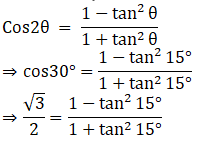Thus, option (C) √3/2 is the correct answer.

36. The value of cos 1° cos 2° cos 3°… cos 179° is
A. 1/√2
B. 0
C. 1
D. –1

Solution:

B. 0

Explanation:

According to the question,

Since cos90° =0

We get,

⇒ cos 1° cos 2° cos 3°… cos90°… cos 179° = 0

Thus, option (B) 0 is the correct answer.

37. If tan θ = 3 and θ lies in third quadrant, then the value of sin θ is
A. 1/√10
B. – 1/√10
C. – 3/√10
D. 3/√10

Solution:

C. – 3/√10

Explanation:

According to the question,

Given that, tan θ = 3 and θ lies in third quadrant

⇒ cot θ = 1/3

We know that,

Cosec2θ = 1+cot2θ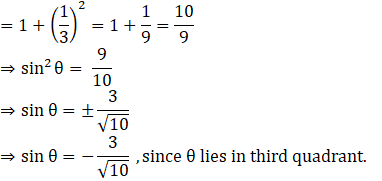Thus, option (C) – 3/√10 is the correct answer.

38. The value of tan 75°– cot 75° is equal to
A. 2√3

B. 2 + √3

C. 2 – √3
D. 1

Solution:

A. 2√3

Explanation:

According to the question,

We have,

tan 75°– cot 75°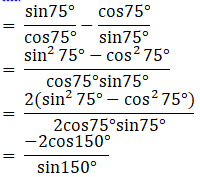= -2cot150°

= -2 cot (180°-30°)

= 2cot30°

=2√3

Thus, option (A) 2√3 is the correct answer.